• 文章目录一、基础概念插值是什么拟合是什么插值和拟合的相同点插值和拟合的不同点二、常用的基本插值方法高次多项式插值拉格朗日多项式插值牛顿插值差商矩阵低次多项式插值（不易震荡）分段线性插值Hermite插值三次...
文章目录摘要（必看）0 基础概念什么是插值插值用途什么是拟合插值和拟合的相同点插值和拟合的不同点1 常用的基本插值方法1.1 多项式插值法1.1.1 拉格朗日多项式插值法多项式插值并不是次数越大越好（龙格现象）分段低次线性插值以提高精度，减小插值误差分段二次插值分段线性插值拉格朗日插值的局限示例1.1.2 牛顿多项式插值法（差商）差商示例低次多项式插值（不易震荡）的其他示例1.1.3 拉格朗日插值和牛顿插值的对比不同相同1.2 考虑导数的插值方法1.2.1Hermite插值分段三次hermite插值1.2.2 三次样条插值示例用插值进行数据预测总结(必看+1)
摘要（必看）
本文特别长（长到需要些摘要·····），这对可读性造成很大负面影响，但我觉得都是插值，放在一起比较方便对比，所以目录逻辑做的比较清晰，实际上又臭又长的本文仅仅讲了两大类插值方法，一类是只考虑最基本插值条件的多项式插值，一类是还要考虑导数的插值，第一类又主要细分为拉格朗日多项式插值和牛顿多项式插值，且分别讲了基于他俩的分段低次插值，第二类主要细分为只考虑一阶导数相等的hermite插值和考虑一二阶导数相等的样条插值，并讲了基于他俩的三次分段插值。所有插值方法都给出了示例，并有图和代码。文末给出了精华总结。
另外，本文参考了很多清风老师的数学建模教程中有关插值的资料（部分公式、代码和文字描述），引用的文字部分都统一用灰框框起来的，清风老师的这套资料和视频讲解特别详细有用，可以在B站找到，虽然要花点小钱，但亲测真的非常值得。

文章太长，滚轮滚起来太慢，可以用crtl + home或者ctrl + end快速到达顶部和底部哦

0 基础概念
什么是插值
求出过已知个有限数据点的近似函数。

插值法是数值分析中最基本的方法之一。 在实际问题中遇到的函数是许许多多的，有的甚至给不出表达式，只供给了一些离散数据，例如，在查对数表时，需要查的数值在表中却找不到，所以只能先找到它相邻的数，再从旁边找出它的更正值，按一定的关系把相邻的数加以更正，从而找出要找的数，这种更正关系事实上就是一种插值。采用不同的插值函数，逼近的效果也不同。

当然啦，到后面你就会知道，已知数据点上取值相同只是插值的最基本条件，是必须满足的基础条件，这个都不满足就不是插值了。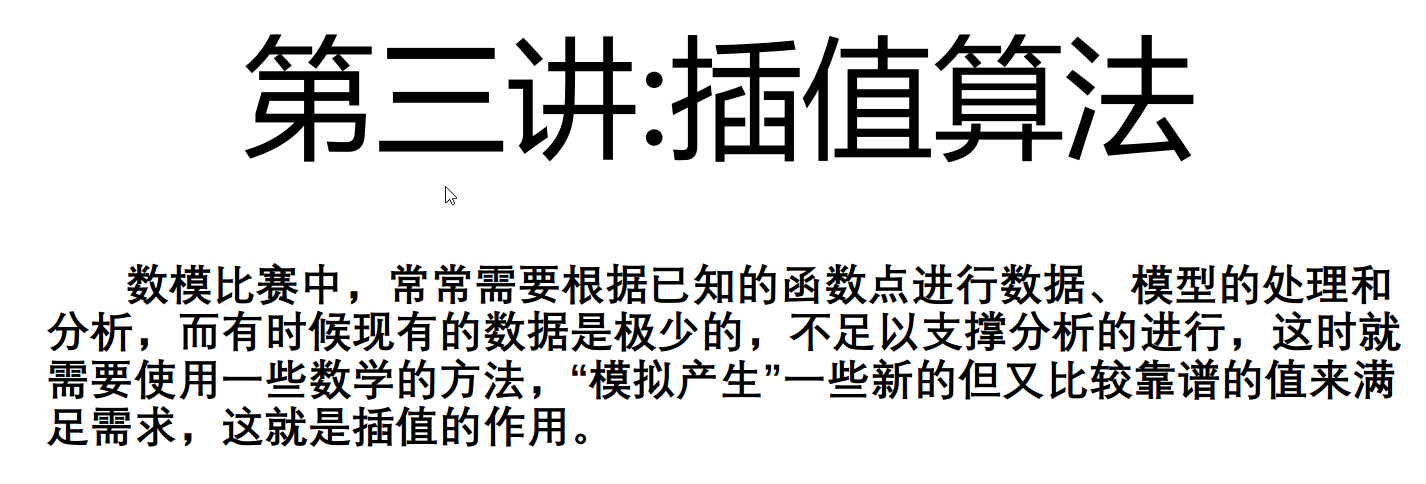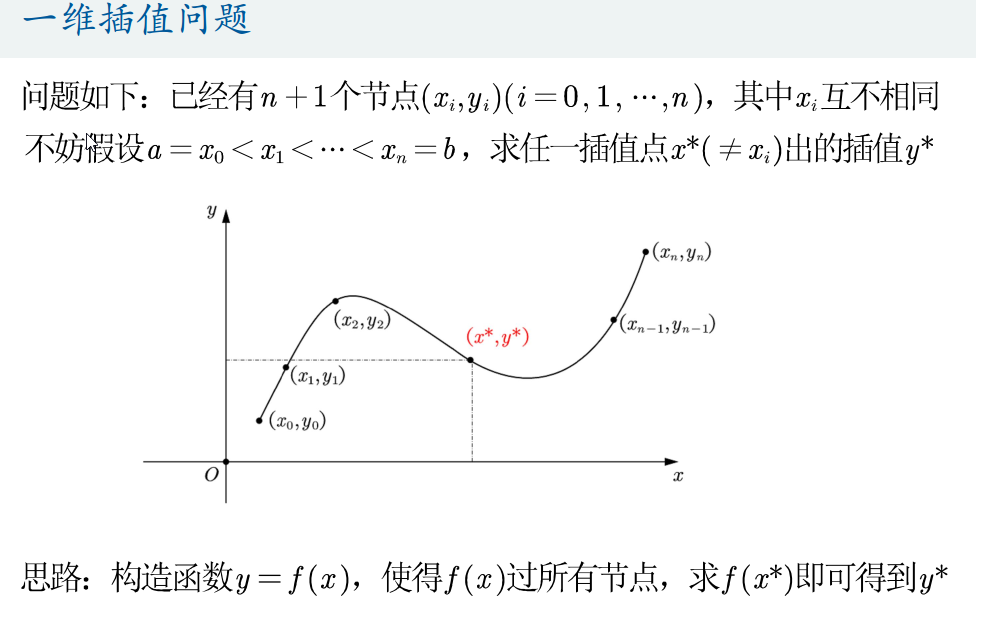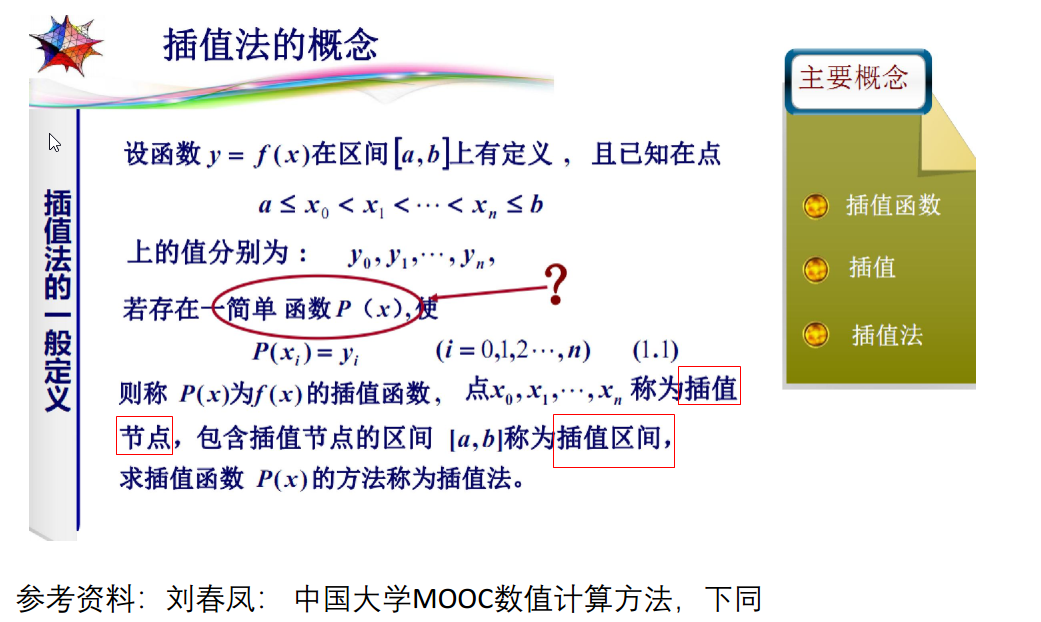插值用途
补全缺失数据
基于已知数据进行预测
什么是拟合
求出一个不要求过已知数据点的近似函数，不要求过那些数据点，只要求在这些点上的总偏差最小。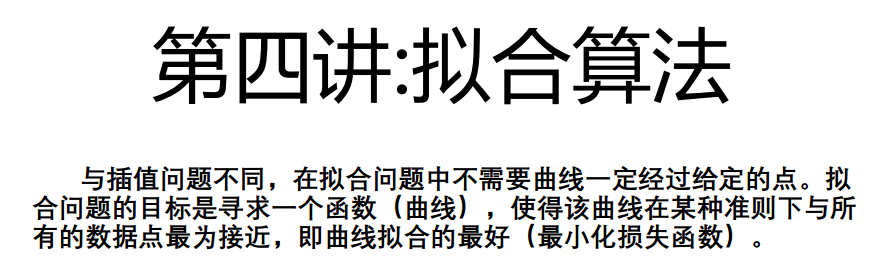插值和拟合的相同点
都是根据一组数据构造一个近似函数
插值和拟合的不同点
近似的要求不同；数学方法完全不同

一个问题到底应该插值还是拟合，有时容易确定有时不能明显看出。
我个人觉得数据点给的很多的时候就不再需要插值了，只需要拟合，看数据的走向趋势即可，因为本身插值就是在数据点太少的情况下看不出数据趋势才需要去生成新的可靠数据点的。另一方面是因为，数据点本身很多的话，多项式插值则次数很高，龙格现象会造成不准确插值。当然这一点可以通过分段低次插值克服，下面会讲

1 常用的基本插值方法
1.1 多项式插值法
不同的多项式插值方法都是去构造一个n次插值多项式

多项式插值是函数插值中最常用的一种形式。在一般的插值问题中，插值条件可以唯一地确定一个次数不超过 的插值多项式。从几何上可以解释为：可以从多项式曲线中找出一些不超过 次的点通过平面上 个不同的点。插值多项式有两种常用的表达式形式，一种是拉格朗日插值多项式，另一种是牛顿插值多项式，此外拉格朗日插值公式与牛顿插值公式永远相等。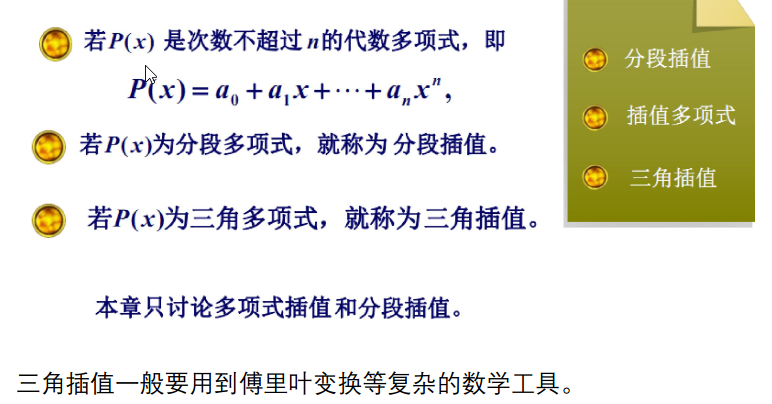只要n+1个节点互不相同，就一定存在唯一的n次多项式使得这n+1个点过这个多项式，厉害！！啊哈哈
若不限制多项式次数为n，就不唯一了哦。也是从证明里可以看出来的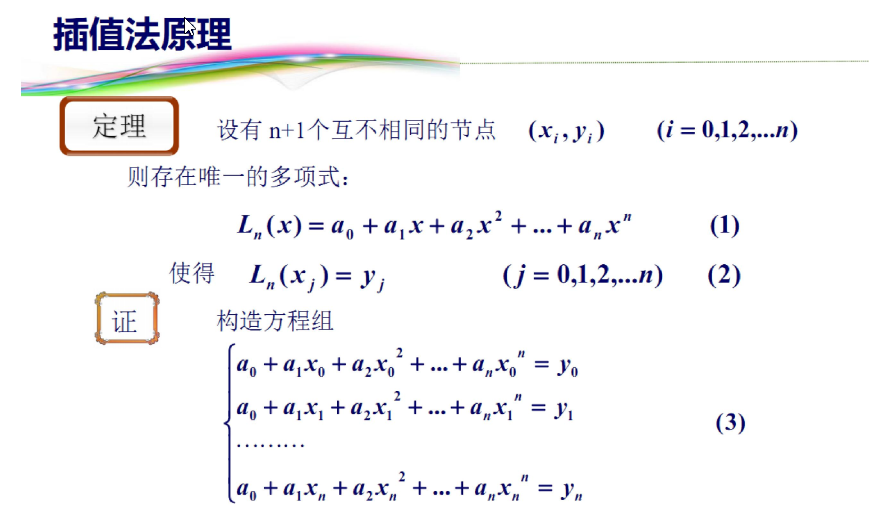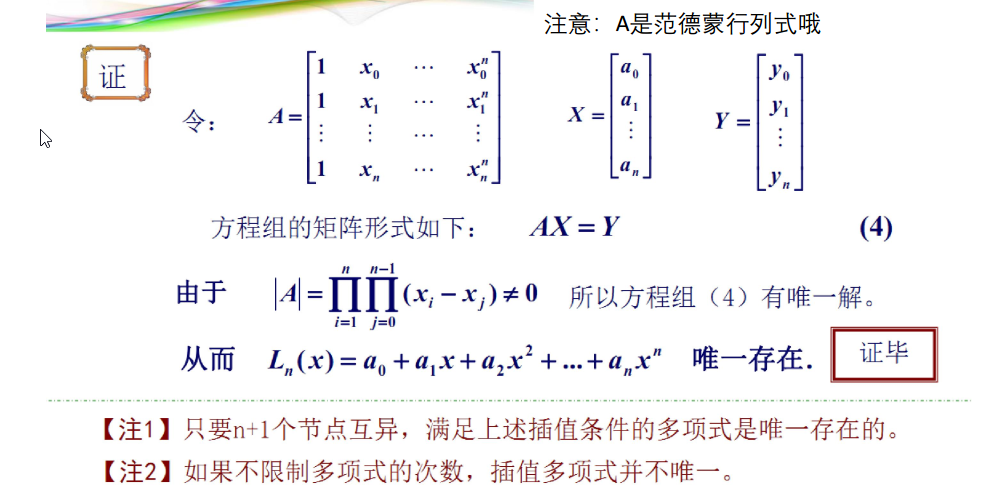1.1.1 拉格朗日多项式插值法
拉格朗日插值多项式是一种特殊的多项式形式，刚好过所有插值节点，是一种很凑巧的多项式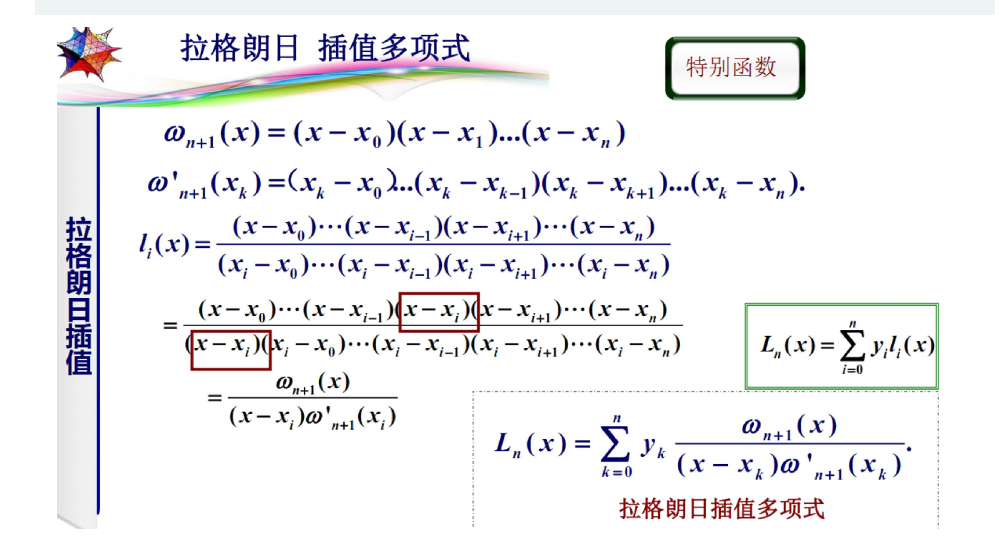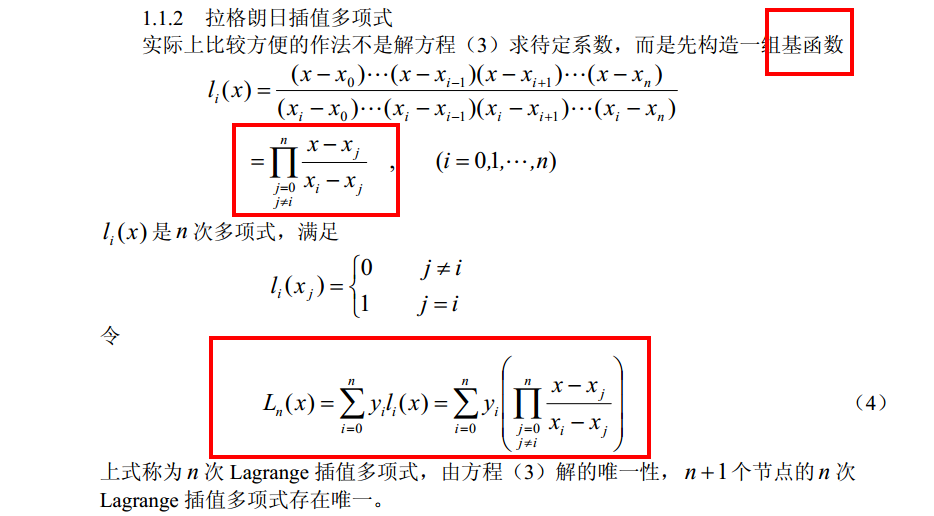例子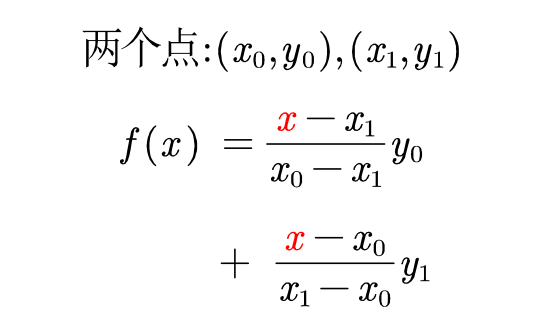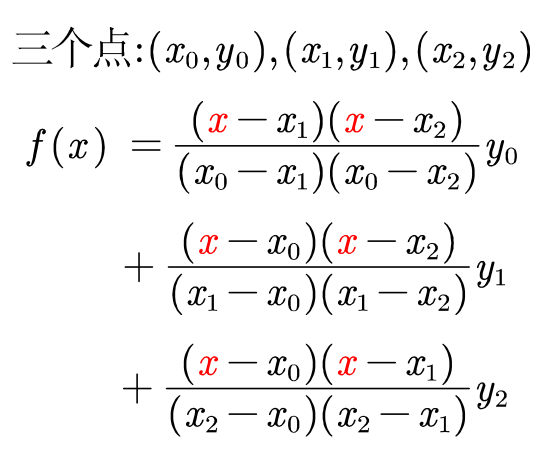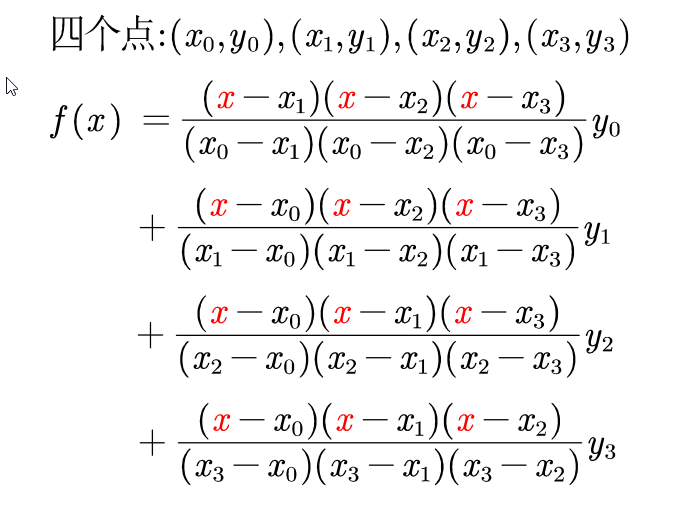所以拉格朗日多项式一共n项，n是已知数据点（插值节点）的数目
多项式插值并不是次数越大越好（龙格现象）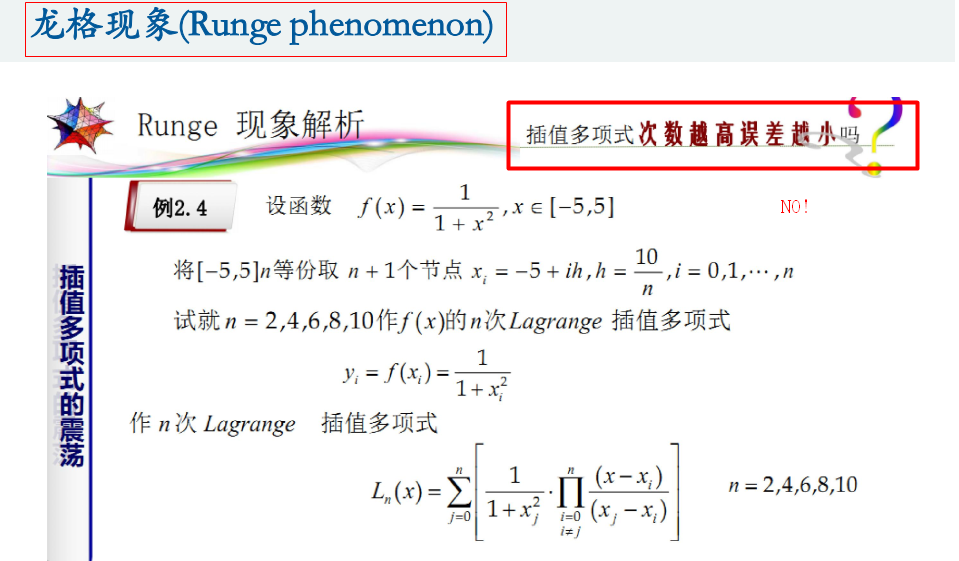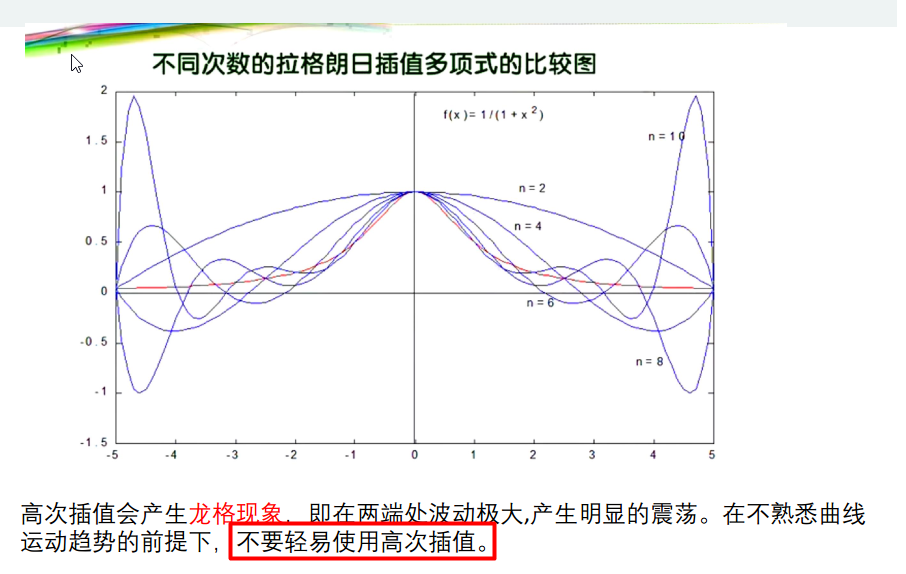分段低次线性插值以提高精度，减小插值误差
分段二次插值
只考虑最近的三个点，进行构造分段的拉格朗日插值多项式，以避免高次多项式插值带来的龙格效应，实现更高的精度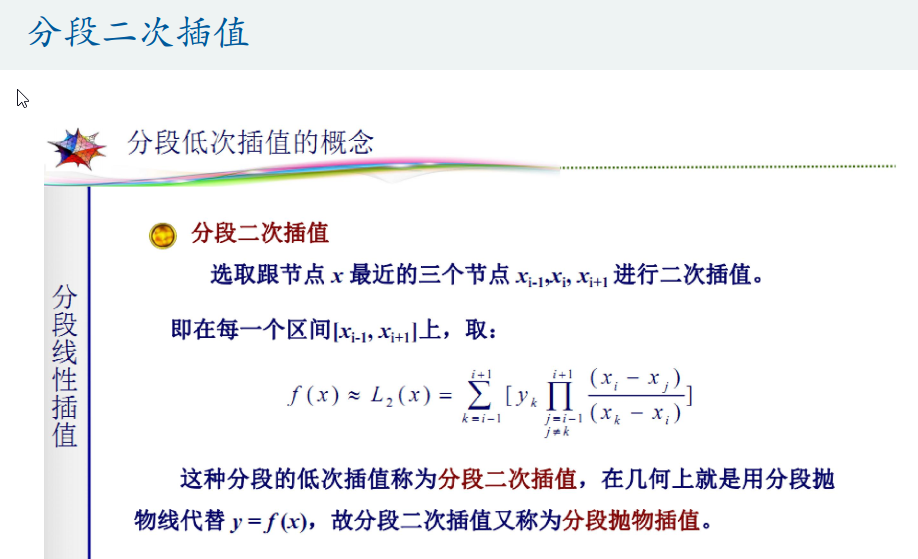这张图的公式有误，应该改为
$f(x)\approx L_2(x)=\sum_{k=i-1}^{i+1}[y_k \prod_{j=i-1 \atop j=\not k}^{i+1}\frac{(x-x_j)}{(x_k-x_j)}]$
实际就是分段线性插值
分段线性插值本质上是多项式插值，而且是加权式的插值方法。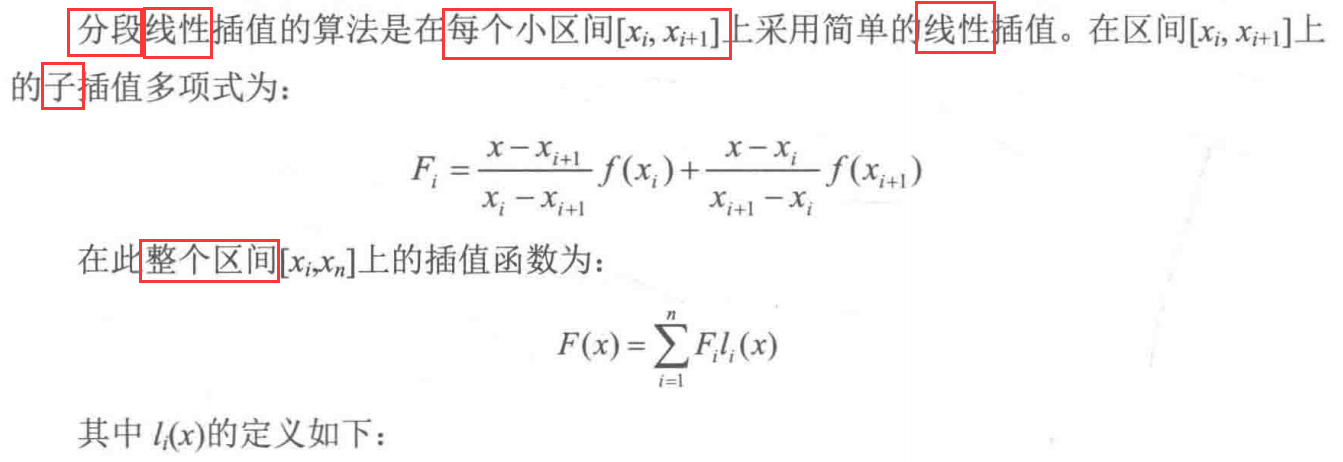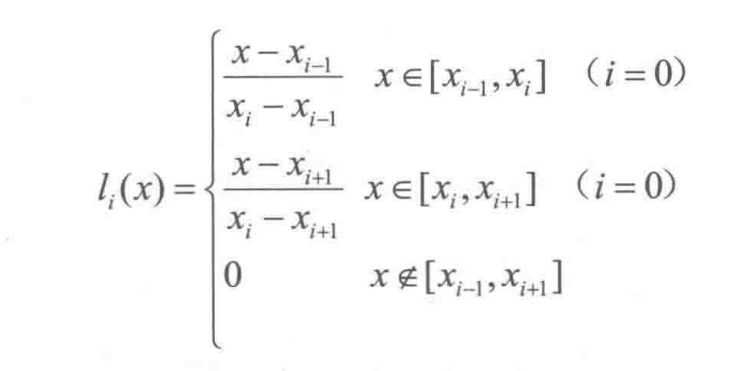第一个图中的$F_i$通分后：
$\frac{x_if(x_{i+1})-x_{i+1}f(x_i)}{x_i-x_{i+1}}+\frac{f(x_i)-f(x_{i+1})}{x_i-x_{i+1}}x$
这其实就是$(x_i,f(x_i))$和$(x_{i+1},f(x_{i+1}))$两点的连线的一次函数
所以分段线性插值的子插值函数没什么高深的，就是每段两个端点的连线。
$l_i(x)$就像是一个权重函数，我们用一个配巨丑手画图的例子来具体看看它为什么是权重
假设有5点已知数据点，$x_1,x_2,\cdots,x_5$,那么子插值函数$F_1,F_2,F_3,F_4$就分别是相邻两点的连线函数
现在对一个未知函数值的取值在$x_1,x_2$之间的点$x_c$进行插值，由于
l_1(x)=\left\{ \begin{aligned} &\frac{x-x_0}{x_1-x_0}&,&x \in[x_0,x_1]\\ &\frac{x-x_2}{x_1-x_2}&,&x \in[x_1,x_2]\\ &0&,& x \notin [x_0,x_2] \end{aligned} \right.\quad l_2(x)=\left\{ \begin{aligned} &\frac{x-x_1}{x_2-x_1}&,&x \in[x_1,x_2]\\ &\frac{x-x_3}{x_2-x_3}&,&x \in[x_2,x_3]\\ &0&,& x \notin [x_1,x_3] \end{aligned} \right.
所以$F(x_c)=F_1(x_c)\frac{x_c-x_2}{x_1-x_2}+F_2(x_c)\frac{x_c-x_1}{x_2-x_1}$
其中$\frac{x_c-x_2}{x_1-x_2}和\frac{x_c-x_1}{x_2-x_1}$分别是$x_c$到$x_2$的距离在$x_1,x_2$之间距离的比例，$x_c$到$x_1$的距离在$x_1,x_2$之间距离的比例
而$F_1(x_c)$则是$x_1,x_2$连线函数上$x_c$对应函数值，即下图图示中的a
$F_2(x_c)$则是$x_2,x_3$连线函数上$x_c$对应函数值，即下图图示中的b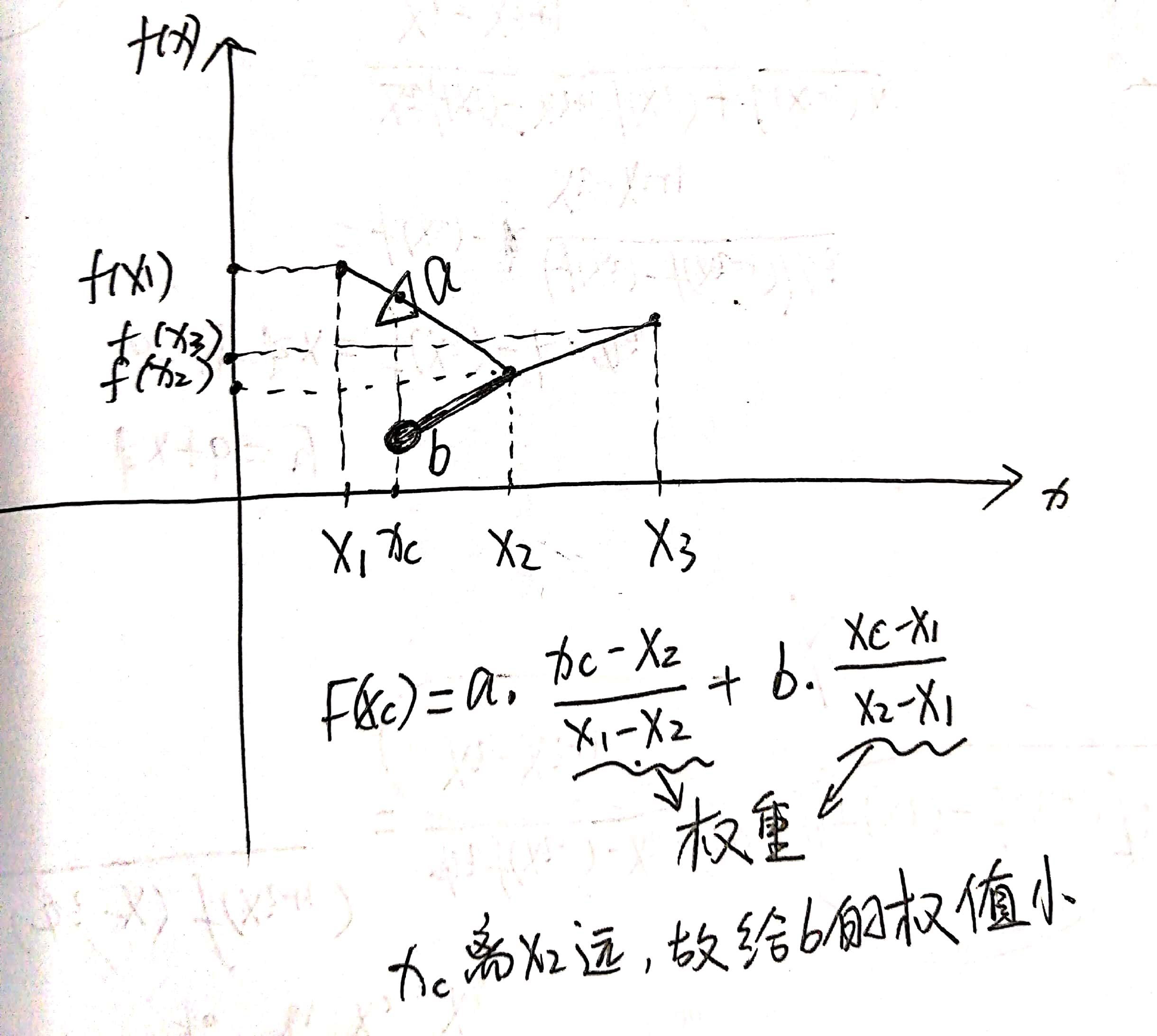n越大，分段越多，插值越好，误差越小
缺点：分段线性插值不能保证在节点处的插值函数的导数的连续性，即不光滑（看后面的示例）
拉格朗日插值的局限
从定义式可看到，每个基函数$l_i(x)$都需要利用所有插值节点来计算，所以一旦新增一个数据点，所有的基函数全部需要重新计算，所以计算量上没有优势，下面介绍的牛顿插值法在这一点上就没有这个缺点示例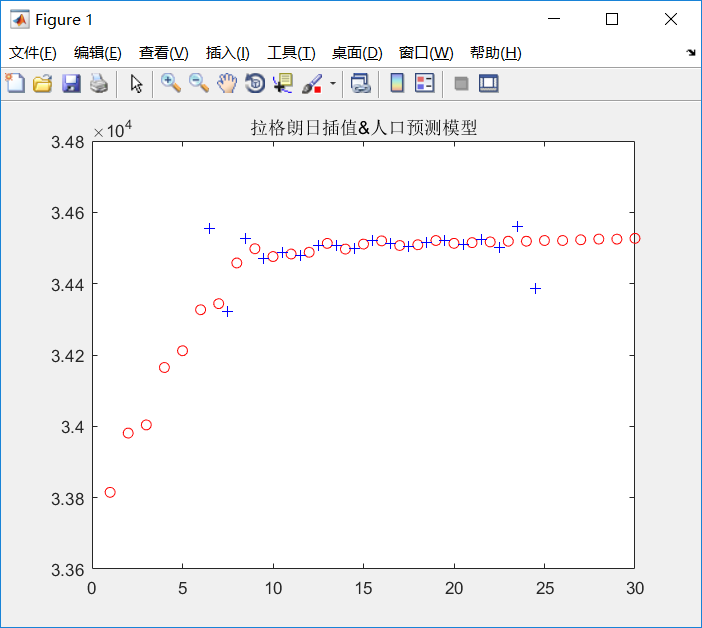人口数据给了30个已知数据点，属于是高次插值，可以看出效果并不理想
代码：
clear all
clc
% 人口数据
T=1:30;  % +1970=年份
P=[33815 33981 34004 34165 34212 34327 34344 34458 34498 34476 34483 34488 34513 34497 34511 34520 34507 34509 34521 34513 34515 34517 34519 34519 34521 34521 34523 34525 34525 34527];%人口
%plot(T,P)% 折线
plot(T,P,'r o')% 只画数据点
hold on
x1=1.5:30.5;
y1=Lagrange(T,P,x1);
plot(x1,y1,'b +')
axis([0 30 33600 34800])
title('拉格朗日插值&人口预测模型')

function y1=Lagrange(x,y,x1);
% x   插值节点
% y   被插值函数在节点x处的函数值
% x1  待插值节点
% y1  待插值节点的插值结果
n=length(x); % 数据点个数
m=length(x1); % 待插值点数
for k=1:m
z=x1(k);
sum=0.0;
for i=1:n
prod = 1.0;
for j=1:n
if j~=i
prod = prod * (z - x(j)) / (x(i) - x(j));
end
end
sum = sum + prod * y(i);
end
y1(k) = sum;
end

1.1.2 牛顿多项式插值法（差商）
插值的思想和目的还是一样的

牛顿插值法的公式是另一种n次插值多项式的构造形式，然而它却克服了拉格朗日插值多项式的缺陷，它的一个显著优势就是每当增加一个插值节点，只要在原牛顿插值公式中增加一项就可形成高一次的插值公式。此外，如果在实际应用中遇到等距分布的插值节点，牛顿插值公式就能得到进一步的简化，从而得到等距节点的插值公式，这样为缩短实际运算时间做出了很大的贡献。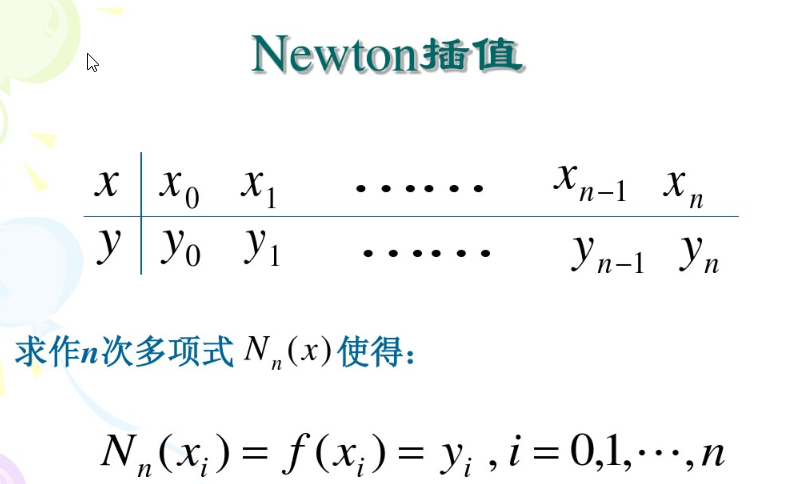差商
一个挺有意思的东西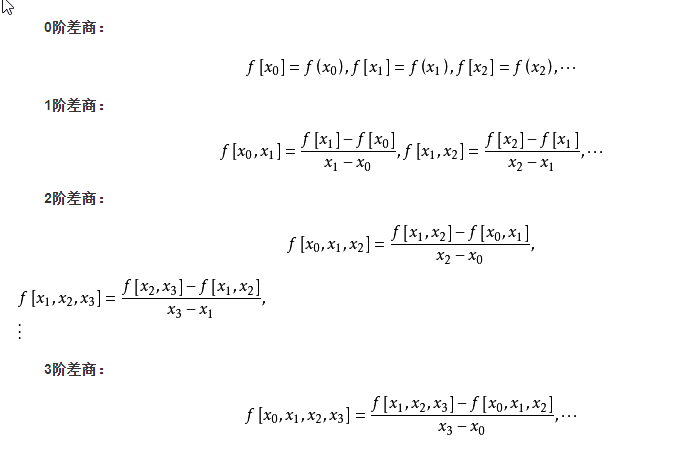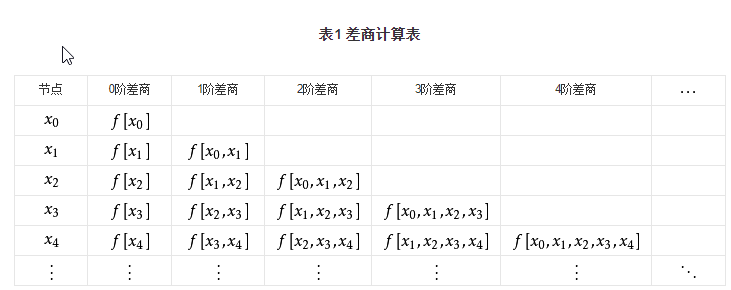##### 差商矩阵
理解差商矩阵是理解牛顿插值代码的灵魂之门
(以5个插值数据点为例，则差商矩阵为5*5)
matlab中索引从1开始，我就保持一致，不从0开始了

y(1)

y(2)
$\frac{y(2)-y(1)}{x(2)-x(1)}$, i.e. $f[x_1,x_2]$

y(3)
$\frac{y(3)-y(2)}{x(3)-x(2)}$, i.e. $f[x_2,x_3]$
$\frac{f[x_2,x_3]-f[x_1,x_2]}{x(3)-x(1)}，i.e. f[x_1,x_2,x_3]$

y(4)
$\frac{y(4)-y(3)}{x(4)-x(3)}$, i.e. $f[x_3,x_4]$
$f[x_2,x_3,x_4]$
$f[x_1,x_2,x_3,x_4]$

y(5)
$\frac{y(5)-y(4)}{x(5)-x(4)}$, i.e. $f[x_4,x_5]$
$f[x_3,x_4,x_5$]
$f[x_2,x_3,x_4,x_5]$
$f[x_1,x_2,x_3,x_4,x_5]$

计算关键代码：
 A(i,j) = (A(i,j-1) - A(i-1,j-1))/(X(i)-X(i-j+1));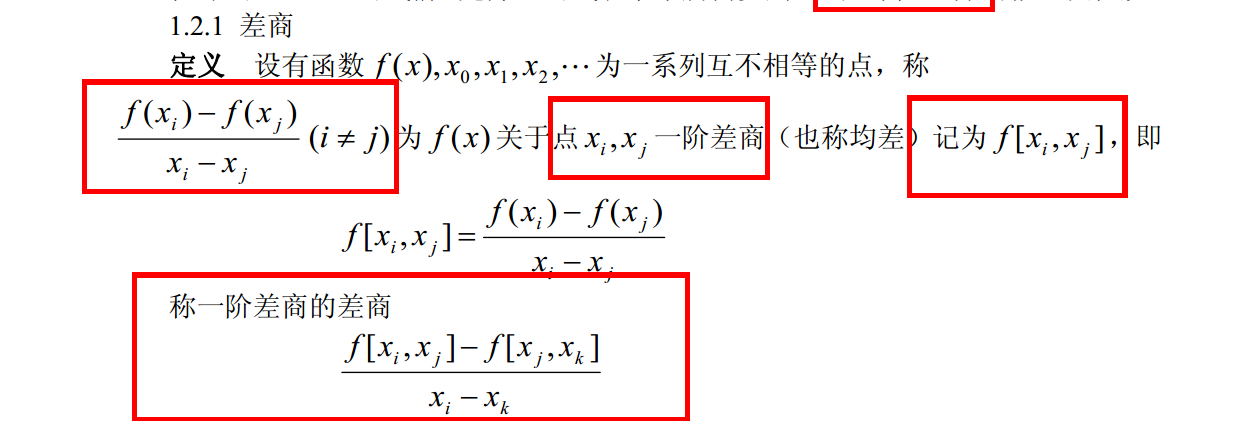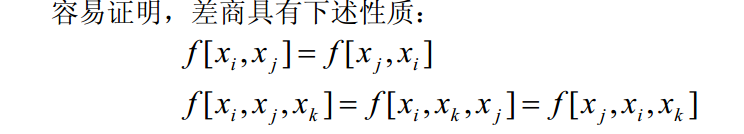示例
此代码被撸了仨小时，参考的一位博主的非常好的代码，把差商用一个矩阵存放，还计算了插值余项，链接
牛顿插值的理论可以在链接里看，讲的很详细
%newton.m
%求牛顿插值多项式、差商、插值及其误差估计的MATLAB主程序
%输入的量:X是n+1个节点(x_i,y_i)(i = 1,2, ... , n+1)横坐标向量，Y是纵坐标向量，
%x是以向量形式输入的m个插值点，M在[a,b]上满足｜f~(n+1)(x)｜≤M
%注：f~(n+1)(x)表示f(x)的n+1阶导数
%输出的量：向量y是向量x处的插值，误差限R，n次牛顿插值多项式L及其系数向量C，
%差商的矩阵A
function[y,R,A,C,L] = newton(X,Y,x,M)
n = length(X);
m = length(x);
for t = 1 : m
z = x(t);
A = zeros(n,n);
A(:,1) = Y';
s = 0.0; p = 1.0; q1 = 1.0; c1 = 1.0;
for j = 2 : n
for i = j : n
A(i,j) = (A(i,j-1) - A(i-1,j-1))/(X(i)-X(i-j+1));
end
q1 = abs(q1*(z-X(j-1))); % 余项计算中的差值连乘项
c1 = c1 * j; % 余项计算中的分母，阶乘
end
C = A(n, n); q1 = abs(q1*(z-X(n)));
for k = (n-1):-1:1
C = conv(C, poly(X(k)));
% poly函数把根转换为求得此根的多项式，X（k）是一个数，所以转换为一次多项式，得到其系数向量
% conv函数的输入是多项式的系数向量时，相当于直接相乘
d = length(C);
C(d) = C(d) + A(k,k);%在最后一维，也就是常数项加上新的差商
end
y(t) = polyval(C,z); % 插值结果
R(t) = M * q1 / c1; % 用M代替f~(n+1)(x)，即f(x)的n+1阶导数，这样求得的余项比真实值略大
end
L = vpa(poly2sym(C),3);% vpa让输出结果系数为小数而非分数,3表示用3位小数

测试脚本：
clear all
clc
X = [0 pi/6 pi/4 pi/3 pi/2];
Y = [0 0.5 0.7071 0.8660 1];
x = linspace(0,pi,50);
M = 1;
[y,R,A,C,L] = newton(X, Y, x, M);
y1 = sin(x);
errorbar(x,y,R,'.g')
hold on
plot(X, Y, 'or', x, y, '.k', x, y1, '-b');
legend('误差','样本点','牛顿插值估算','sin(x)');

这里已知数据点少，多项式次数低，插值效果就比较好
插值效果：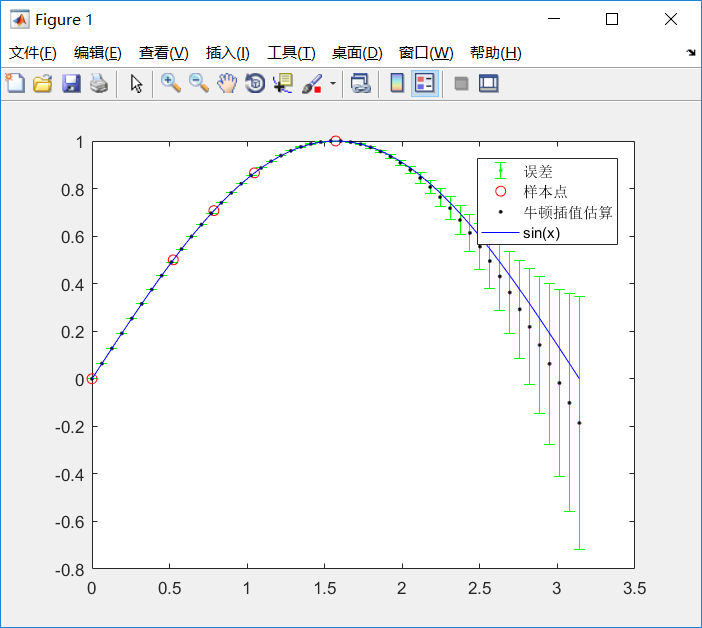计算出的牛顿插值多项式，多项式系数，差商矩阵：
>> C
C =
0.0290   -0.2048    0.0217    0.9955         0
>> L
L =
0.029*x^4 - 0.205*x^3 + 0.0217*x^2 + 0.996*x
>> A
A =
0         0         0         0         0
0.5000    0.9549         0         0         0
0.7071    0.7911   -0.2086         0         0
0.8660    0.6070   -0.3516   -0.1365         0
1.0000    0.2559   -0.4469   -0.0910    0.0290

另一套数据的插值结果：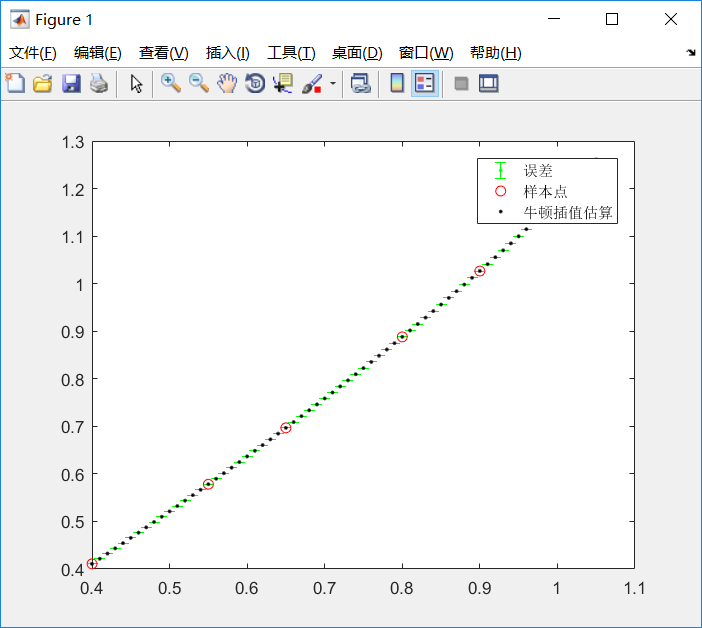代码：
X=[0.4 0.55 0.65 0.80 0.90 1.05];
Y=[0.41075 0.57815 0.69675 0.88811 1.02652 1.25386];
x=0.4:0.01:1.05;
M = 1;
[y,R,A,C,L] = newton(X, Y, x, M);
errorbar(x,y,R,'.g')
hold on
plot(X, Y, 'or', x, y, '.k');
legend('误差','样本点','牛顿插值估算');

用于人口数据插值：
惨不忍睹的结果······
所以并没有一种插值可以通用，完美拟合任何数据，要选择适合数据的插值方式
这个人口数据呈明显的对数函数趋势，要用对数型函数去拟合（见这篇博客）。而**不能插值！！！**除非用于预测，某年数据还说得过去
这里的数据点有30个，很多，牛顿插值得到了29次多项式, 共30个系数，高次多项式的函数图像很容易震荡，下图也可以看出插值数据的震荡性，这使得得到的插值函数并不光滑，而人口数据本身是很光滑的对数形状的曲线，这无法保证插值函数处处收敛于被插值函数（就是原数据点真正遵循的那个函数），这是高次多项式插值的通病。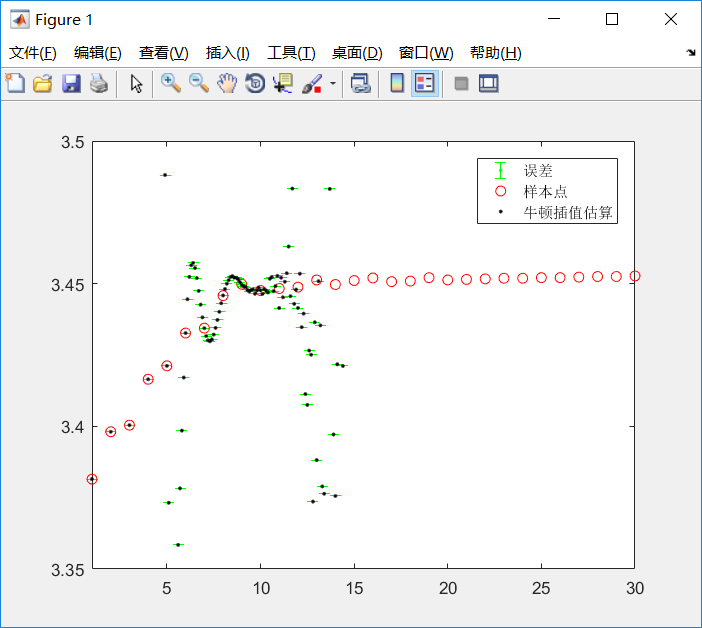代码：
clear all
clc
% 人口数据
T=1:30;  % +1970=年份
P=[33815 33981 34004 34165 34212 34327 34344 34458 34498 34476 34483 34488 34513 34497 34511 34520 34507 34509 34521 34513 34515 34517 34519 34519 34521 34521 34523 34525 34525 34527];%人口
P=P/10000;
M = 1;
x=1:.1:30;
[y,R,A,C,L] = newton(T, P, x, M);
errorbar(x,y,R,'.g')
hold on
axis([1 30 3.35 3.50])
plot(T, P, 'or', x, y, '.k');
legend('误差','样本点','牛顿插值估算');

低次多项式插值（不易震荡）的其他示例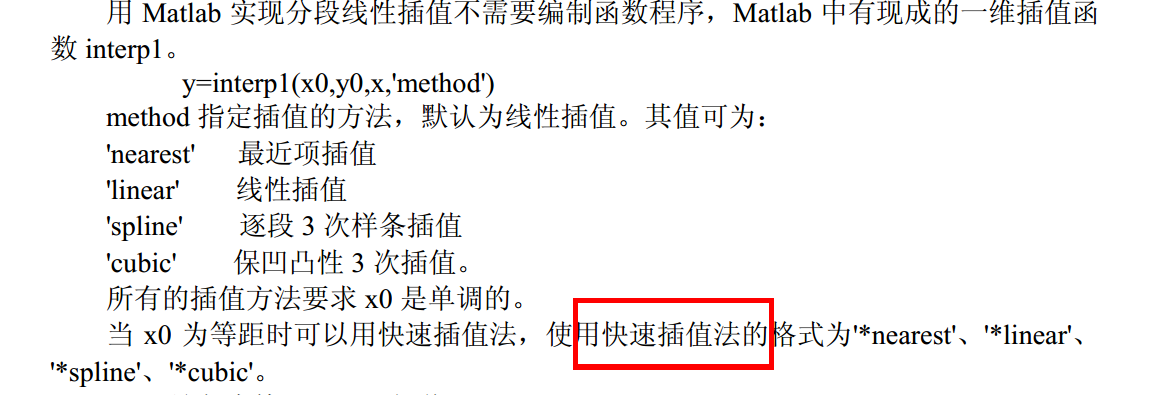保凹凸性即hermite插值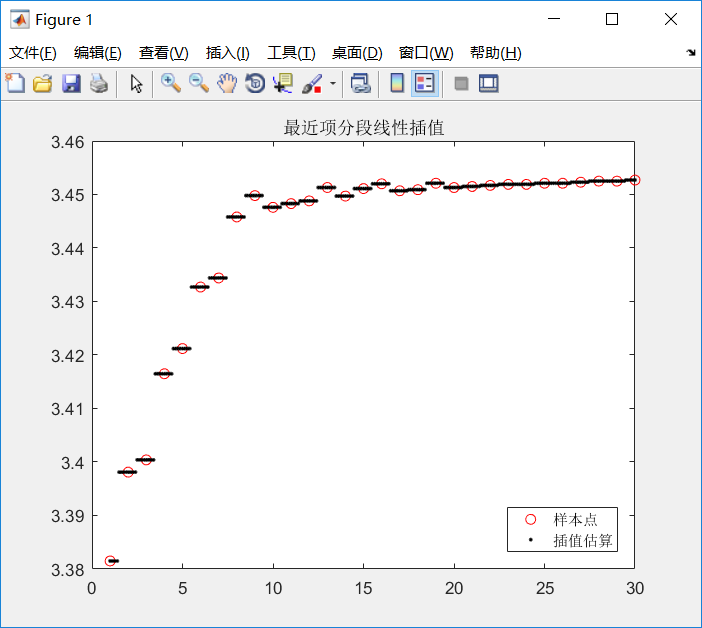最差无疑了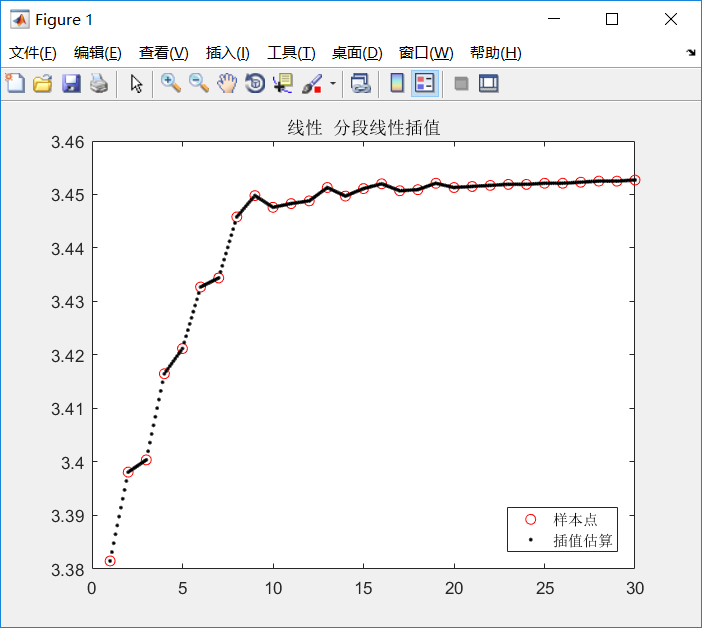分段线性插值的子插值函数都是一次的，从图像上也可以看得出来，一段一段的，很不光滑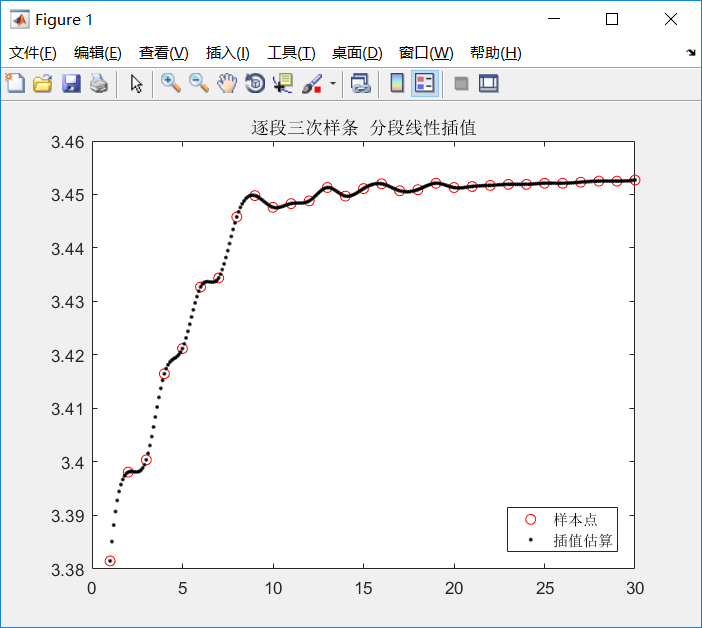三次样条的结果明显要光滑很多，子插值函数都是三次的，还考虑了一二阶导数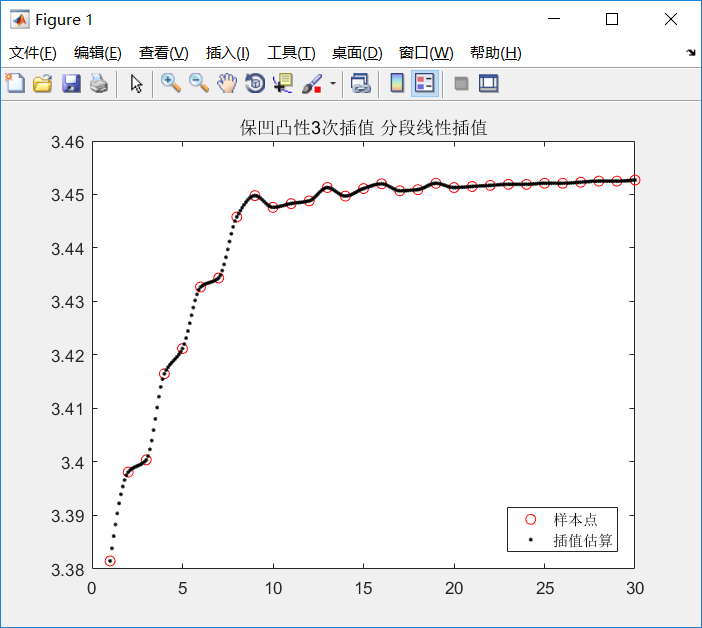代码：
clear all
clc
% 人口数据
T=1:30;  % +1970=年份
P=[33815 33981 34004 34165 34212 34327 34344 34458 34498 34476 34483 34488 34513 34497 34511 34520 34507 34509 34521 34513 34515 34517 34519 34519 34521 34521 34523 34525 34525 34527];%人口
P=P/10000;
x=1:.1:30;
%y=interp1(T,P,x,'*nearest') ;
%y=interp1(T,P,x,'*linear') ;
%y=interp1(T,P,x,'*spline') ;
y=interp1(T,P,x,'*cubic') ;
axis([1 30 3.35 3.50])
plot(T, P, 'or', x, y, '.k');
legend('样本点','插值估算','Location','southeast');
%title('最近项分段线性插值')
%title('线性  分段线性插值')
% title('逐段三次样条  分段线性插值')
title('保凹凸性3次插值 分段线性插值')

% 'north' 坐标轴中的顶部
% 'south' 坐标轴中的底部
% 'east' 坐标轴中的右侧区域
% 'west' 坐标轴中的左侧区域
% 'northeast' 坐标轴中的右上角（二维坐标轴的默认值）
% 'northwest' 坐标轴中的左上角
% 'southeast' 坐标轴中的右下角
% 'southwest' 坐标轴中的左下角
% 'northoutside' 坐标轴的上方
% 'southoutside' 坐标轴的下方
% 'eastoutside' 到坐标轴的右侧
% 'westoutside' 到坐标轴的左侧
% 'northeastoutside' 坐标轴外的右上角（三维坐标轴的默认值）
% 'northwestoutside' 坐标轴外的左上角
% 'southeastoutside' 坐标轴外的右下角
% 'southwestoutside' 坐标轴外的左下角
% 'best' 坐标轴内与绘图数据冲突最少的地方
% 'bestoutside' 到坐标轴的右侧
% 'none' 由 Position 属性决定。可使用 Position 属性在自定义位置显示图例。

尝试用在样本数据点少的情况：
对sin函数插值（前面用牛顿插值取得了不错的效果），可以看到和真实sin函数在后面差距越来越大。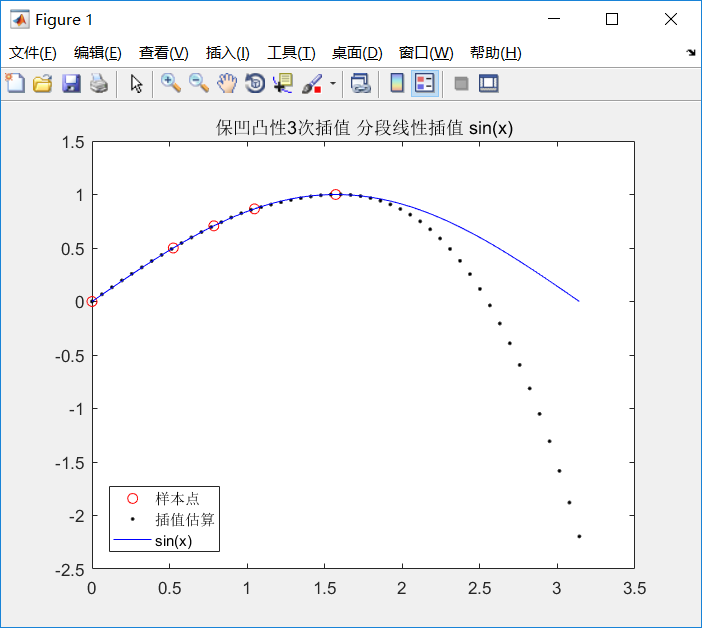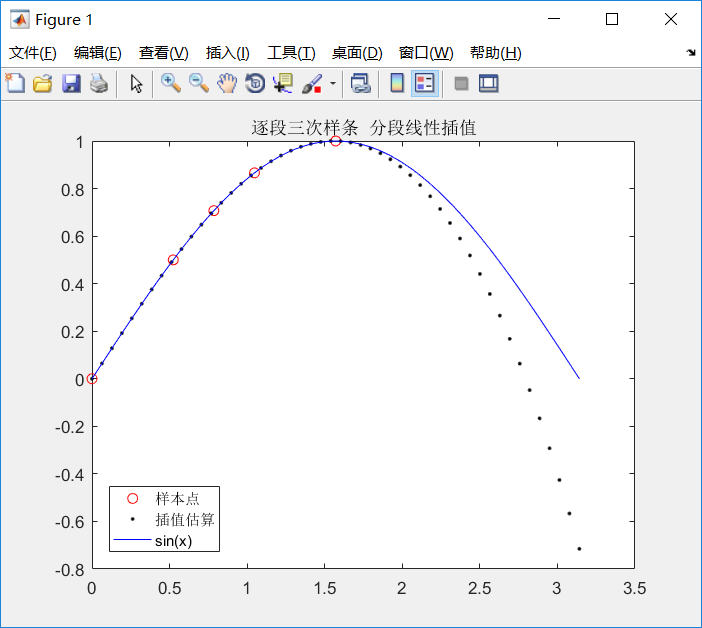这时候最近项线性插值是完全不能用的：
可以看出最近项分段线性插值只能用于数据点非常非常多的情况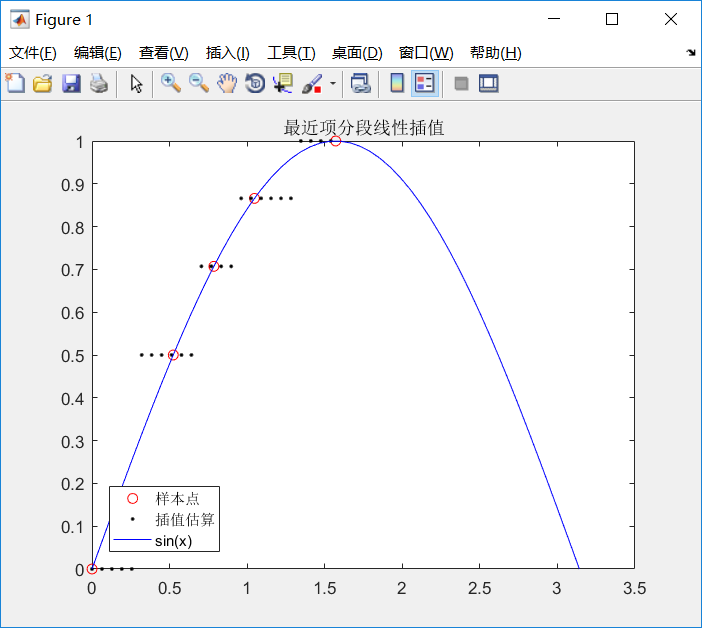linear分段线性插值也不适合数据点少：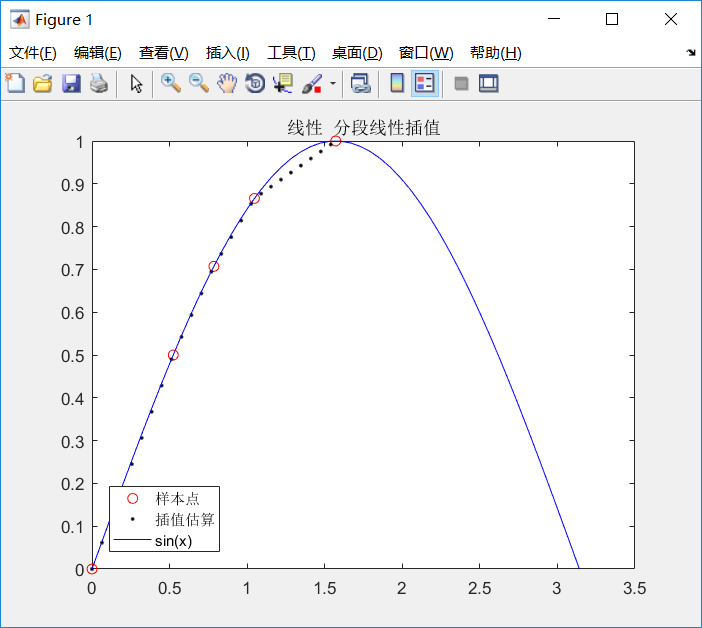1.1.3 拉格朗日插值和牛顿插值的对比
不同
前者不具有继承性导致计算量大
后者有继承性，数据点增多只需要在原来的多项式基础上增加几项，计算量要小一些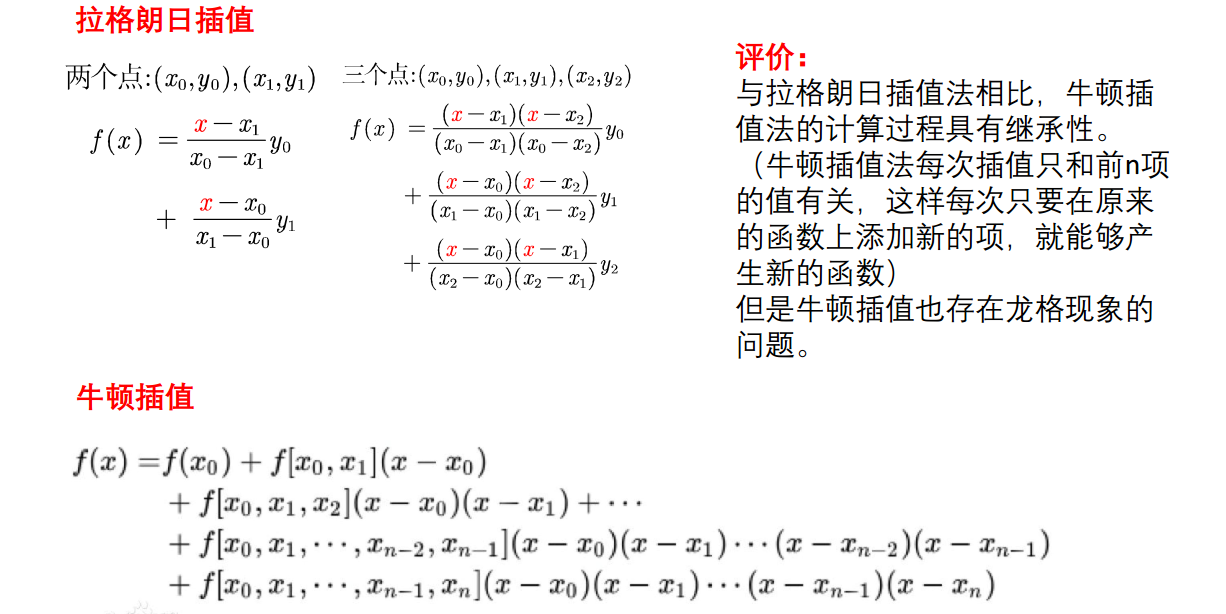相同
都存在龙格效应
都没有考虑导数也要相同，所以可能不能模拟出被插值函数的形态，所以就引入了后面的考虑导数的插值法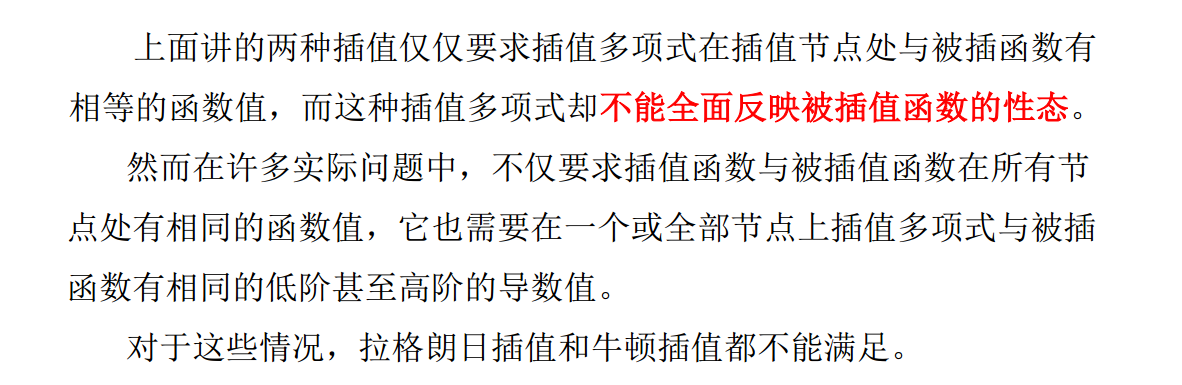1.2 考虑导数的插值方法
1.2.1Hermite插值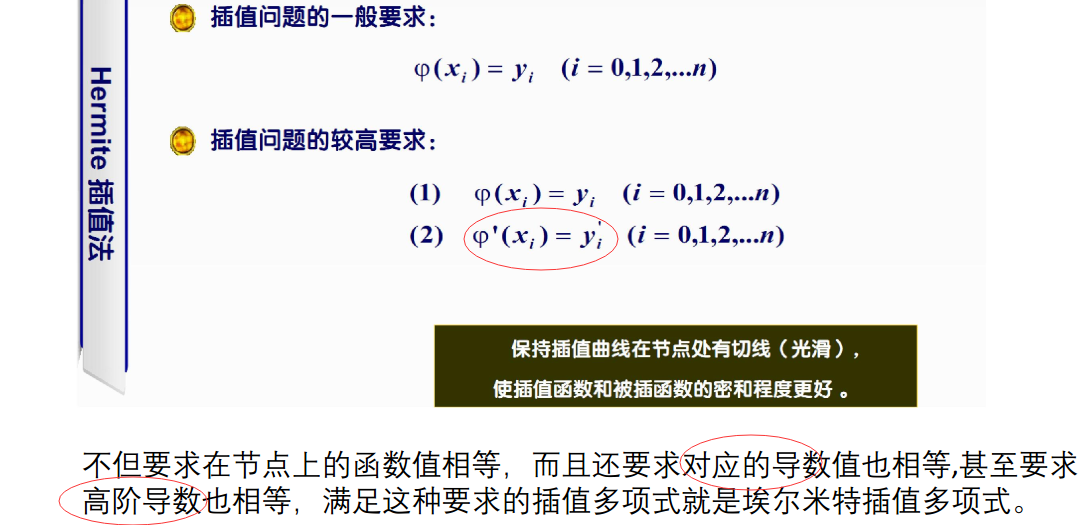分段三次hermite插值
有前途，就用这个了，比分段线性插值好呀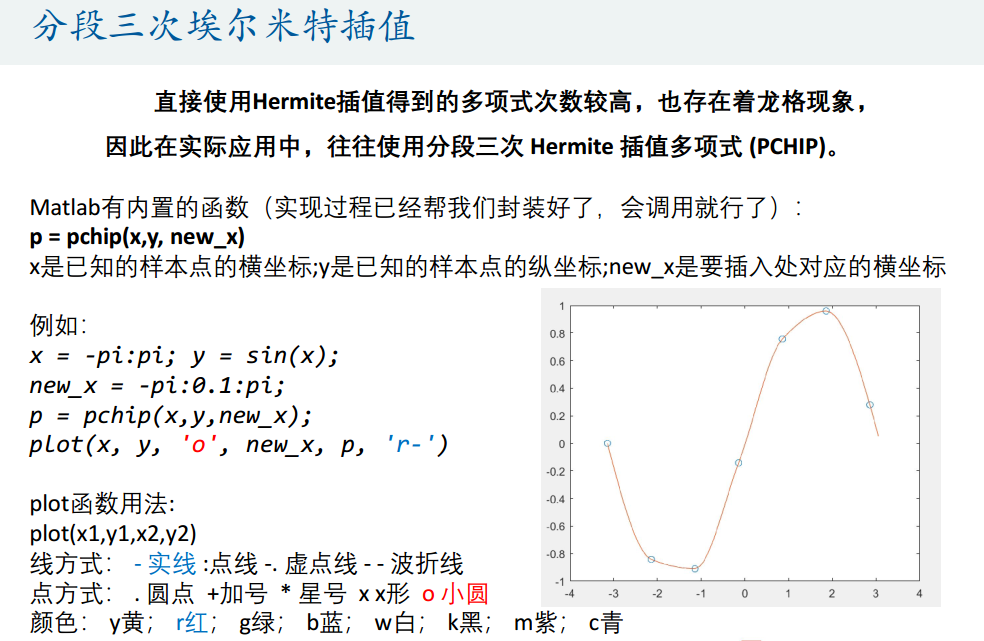优点：关于插值函数和被插函数的贴合程度，埃尔米特插值比多项式的好。
缺点：埃尔米特插值只有在被插值函数在插值节点处的函数值和导数值已知时才可以使用，而这在实际问题中是无法实现的，因为在一般情况下我们是不可能也没必要知道函数在插值节点处的导数值。因此成为能否运用埃尔米特插值的一个重要因素就是：我们知不知道插值函数在节点处的导数值。

1.2.2 三次样条插值
定义
每个子区间的多项式函数是三次的，所以名字里有“三次”，就像前面拉格朗日插值的分段二次插值的每个子区间的插值函数是二次的一样
整个插值函数曲线由分段三次曲线并接而成，并且在连接点也就是样点上必须要二阶连续可导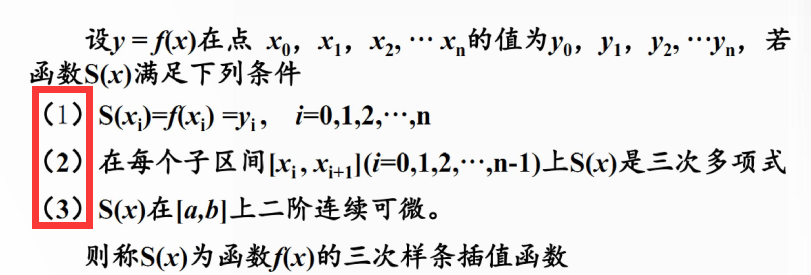n+1个数据点，共有n个分段多项式
相邻两个子多项式之间必须在函数值，一阶导数，二阶导数上都相等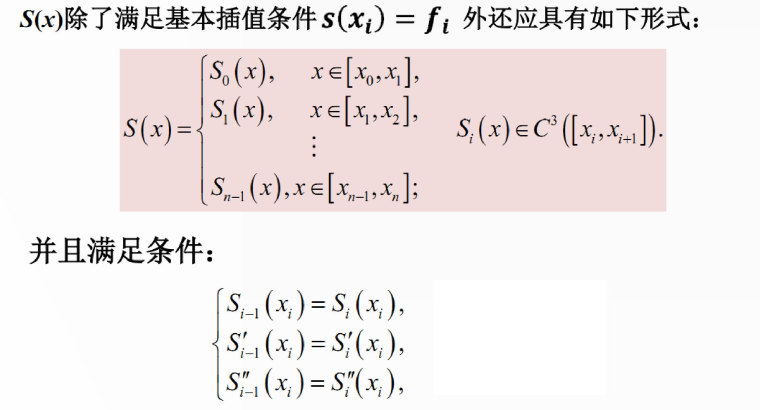对比发现，分段三次hermite插值只考虑了一阶导数，分段三次样条考虑了一阶导数和二阶导数，所以后者得到的结果更加精确
示例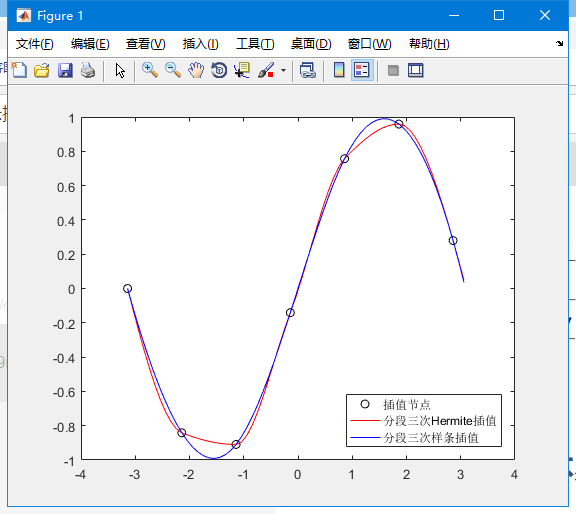代码
x = -pi:pi;
y = sin(x);
x_ = -pi:.1:pi;
p1 = pchip(x,y,x_); % 分段三次Hermite插值
p2 = spline(x,y,x_); % 分段三次样条插值
plot(x,y,'ko',x_,p1,'r-',x_,p2,'b-')
legend('插值节点','分段三次Hermite插值','分段三次样条插值','location','southeast')

可见，三次样条的结果更加光滑，也更接近sin函数本身的图像
用插值进行数据预测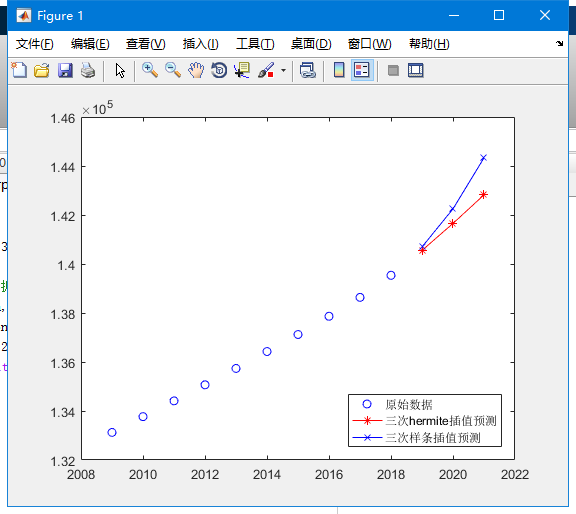代码
% 用插值进行预测
% 2009-2018 10年的人口数据
population=[133126,133770,134413,135069,135738,136427,137122,137866,138639, 139538];
year = 2009:2018;
% 预测 2019-2021三年的人口数据
p1 = pchip(year, population, 2019:2021);
p2 = spline(year, population, 2019:2021);
plot(year, population,'bo',2019:2021,p1,'r*-',2019:2021,p2,'bx-')
legend('原始数据','三次hermite插值预测','三次样条插值预测','location','southeast')

总结(必看+1)

拉格朗日插值、牛顿插值、分段线性插值、分段三次Hermite插值和分段三次样条插值具有不同的优势和适用范围，对于方法的选择至关重要，我们需要对它们进行差异化的了解与认知。

分段插值函数比如分段线性插值、3次分段hermite和三次分段样条插值函数在每个单元区间上收敛性强，数值稳定性好且易于计算机编程实现

一般来说，不要直接使用多项式插值，尤其是数据点较多（n>7）时，高次多项式震荡很厉害；
分段多项式插值可以避免震荡带来的误差；
分段多项式插值又不如分段hermite，因为后者考虑了一阶导数；
分段hermite又不如分段三次样条，因为后者除了一阶导数，还考虑了二阶导数，更好地保持数据的光滑性和连续性，减少信息量的损失。

回顾上面的所有示例图，一般来说，使用逐段三次样条可以获得最优插值结果，由于我们不知道数据本身的分布，所以分段三次hermite也可以尝试。其他的当然也是可以使用的，根据自己的问题自己判断吧

一般来说，使用逐段三次样条可以获得最优插值结果
一般来说，使用逐段三次样条可以获得最优插值结果
一般来说，使用逐段三次样条可以获得最优插值结果
还是要分情况的，最近发现，数据量很大时，比如一条数据向量三千多维，给一千个索引，得到的插值结果在两端会出现强烈的龙格现象，很大的甩尾swings，也是一个棘手的山芋
如果给的索引不是从1开始，linear 和nearest竟然不给两端插值！！！！！我也是服气，整出NAN玩儿
K>> interp1([3 5 7],[4 3 6],1:9,'linear')

ans =

NaN       NaN    4.0000    3.5000    3.0000    4.5000    6.0000       NaN       NaN

K>> interp1([3 5 7],[4 3 6],1:9,'nearest')

ans =

NaN   NaN     4     3     3     6     6   NaN   NaN

K>> interp1([3 5 7],[4 3 6],1:9,'cubic')
警告: 在以后的版本中将会更改 INTERP1(...,'CUBIC')。请改用 INTERP1(...,'PCHIP')。
> In interp1>sanitycheckmethod (line 265)
In interp1>parseinputs (line 401)
In interp1 (line 80)
In feature_extract (line 393)

ans =

11.0000    6.3750    4.0000    3.1250    3.0000    3.8750    6.0000    8.6250   11.0000

K>> interp1([3 5 7],[4 3 6],1:9,'spline')

ans =

9     6     4     3     3     4     6     9    13



展开全文matlab
• ## 多项式插值

千次阅读 2015-10-09 15:12:25
给定n+1n+1个点{(xi,yi)}ni=0\{(x_i,y_i)\}^{n}_{i=0}（称为插值点），所谓多项式插值就是找到一个多项式（称为插值多项式） y=P(x)=akxk+ak−1xk−1+⋯+a1x+a0y=P(x)=a_kx^k+a_{k-1}x^{k-1}+\cdots+a_1x+a_0 使得...
多项式插值

1、多项式插值的定义

给定n+1$n+1$个点{(xi,yi)}ni=0$\{(x_i,y_i)\}^{n}_{i=0}$（称为 插值点），所谓 多项式插值 就是找到一个多项式（称为 插值多项式）
y=P(x)=akxk+ak−1xk−1+⋯+a1x+a0y=P(x)=a_kx^k+a_{k-1}x^{k-1}+\cdots+a_1x+a_0
使得它满足条件
yi=P(xi)，其中i=0,1,…,ny_i=P(x_i)，其中i=0,1,\dots,n
也就是说，多项式y=P(x)$y=P(x)$的图像要经过给定的n+1$n+1$个点。

在实际应用中，这些插值点可能来自某次实验测量所得的数据，也可能来自某个复杂函数y=f(x)$y=f(x)$的值。通过计算插值多项式，我们可以找到这些实验数据间的规律，或者使用简单的多项式函数y=P(x)$y=P(x)$来近似复杂的函数y=f(x)$y=f(x)$。

2、插值多项式的存在唯一性和误差

满足以上条件的多项式总是存在的。特别地，我们可以证明：

定理一：给定n+1$n+1$个点{(xi,yi)}ni=0$\{(x_i,y_i)\}^{n}_{i=0}$，若xi$x_i$两两不同，则存在 唯一 一个次数不超过n$n$的多项式y=P(x)$y=P(x)$，使得yi=P(xi)$y_i=P(x_i)$（i=0,1,…,n$i=0,1,\dots,n$）成立。
证明：利用范德蒙德矩阵和代数学基本定理即得。

注意：在本文中我们均假定xi$x_i$互不相同。

当yi$y_i$的值来自某个函数y=f(x)$y=f(x)$，且f(x)$f(x)$具有n+1$n+1$阶连续导数时，下面的定理可以用来计算多项式插值的（截断）误差。

定理二：给定n+1$n+1$个点{(xi,yi)}ni=0$\{(x_i,y_i)\}^{n}_{i=0}$，其中yi=f(xi)$y_i=f(x_i)$，进一步假设函数f(x)$f(x)$具有n+1$n+1$阶连续导数，则插值多项式P(x)$P(x)$的误差R(x)$R(x)$为
R(x)=f(x)−P(x)=f(n+1)(ξ)(n+1)!(x−x0)(x−x1)⋯(x−xn)R(x)=f(x)-P(x)=\frac{f^{(n+1)}(\xi)}{(n+1)!}(x-x_0)(x-x_1)\cdots(x-x_n)
其中min{xi}≤ξ≤max{xi}$\min\{x_i\}\leq\xi\leq\max\{x_i\}$。

3、插值多项式的计算

给定n+1$n+1$个点{(xi,yi)}ni=0$\{(x_i,y_i)\}^{n}_{i=0}$，计算插值多项式的主要方法有：直接法、拉格朗日多项式插值和牛顿多项式插值。下面我们分别介绍这三种方法。（注意，根据定理一，这三种方法得到的插值多项式在理论上说应该是一致的，而且误差也相同。）

3.1 直接法

根据定理一，假设插值多项式为
y=P(x)=anxn+an−1xn−1+⋯+a1x+a0y=P(x)=a_nx^n+a_{n-1}x^{n-1}+\cdots+a_1x+a_0
由插值条件yi=P(xi)$y_i=P(x_i)$（i=0,1,…,n$i=0,1,\dots,n$)，我们得到关于系数an,an−1,…,a1,a0$a_n,a_{n-1},\dots,a_1,a_0$的线性方程组
⎛⎝⎜⎜⎜⎜⎜⎜⎜⎜xn0xn1⋮xnn−1xnnxn−10xn−11⋮xn−1n−1xn−1n⋯⋯⋱⋯⋯x0x1⋮xn−1xn11⋮11⎞⎠⎟⎟⎟⎟⎟⎟⎟⎟⎛⎝⎜⎜⎜⎜⎜⎜⎜anan−1⋮a1a0⎞⎠⎟⎟⎟⎟⎟⎟⎟=⎛⎝⎜⎜⎜⎜⎜⎜⎜y0y1⋮yn−1yn⎞⎠⎟⎟⎟⎟⎟⎟⎟\left(\begin{array}{lllll}
x^n_0 & x^{n-1}_0 & \cdots & x_0 & 1\\
x^n_1 & x^{n-1}_1 & \cdots & x_1 & 1\\
\vdots & \vdots & \ddots & \vdots & \vdots \\
x^n_{n-1} & x^{n-1}_{n-1} & \cdots & x_{n-1} & 1\\
x^n_n & x^{n-1}_n & \cdots & x_n & 1\\
\end{array}\right)
\left(\begin{array}{l}
a_n \\ a_{n-1}\\ \vdots \\ a_1 \\ a_0\\
\end{array}\right)=\left(\begin{array}{l}
y_0 \\ y_1\\ \vdots \\ y_{n-1} \\ y_n\\
\end{array}\right)
通过求解这个线性方程组，即得到插值多项式。

优点：直接，性质一目了然。
缺点：待求解的线性方程组的系数矩阵为 范德蒙德 (Vandermonde)矩阵，它是一个病态矩阵，这使得在实际求解方程组时将产生很大的误差。

3.2 拉格朗日多项式插值

拉格朗日(Lagrange)多项式插值的原理是：先构造一组 拉格朗日基函数 Li(x)$L_i(x)$（i=0,1,…,n$i=0,1,\dots,n$），这些基函数为次数不超过n$n$的多项式，且具有性质
Li(xj)=δij={10当 i=j当 i≠jL_i(x_j)=\delta_{ij}=\begin{cases}1 & \mbox{当 }i=j\\0 & \mbox{当 }i\neq j\end{cases}
然后将这些基函数做线性组合，得到拉格朗日插值多项式
L(x)=∑i=0nLi(x)yiL(x)=\sum^n_{i=0}L_i(x)y_i
容易验证，多项式L(x)$L(x)$满足插值条件yi=L(xi)$y_i=L(x_i)$（i=0,1,…,n$i=0,1,\dots,n$)。

拉格朗日基函数Li(x)$L_i(x)$的构造如下：

由基函数的性质，当j∈{0,1,…,n}∖{i}$j\in\{0,1,\dots,n\}\setminus\{i\}$时Li(xj)=0$L_i(x_j)=0$，即xj$x_j$为Li(x)$L_i(x)$的零点，可以假设
Li(x)=K⋅∏j≠i(x−xj)L_i(x)=K\cdot \prod_{j\neq i}(x-x_j)
其中，K$K$为待定系数。再由Li(xi)=1$L_i(x_i)=1$，得到
Li(xi)=K⋅∏j≠i(xi−xj)=1L_i(x_i)=K\cdot \prod_{j\neq i}(x_i-x_j)=1
从而得到
K=1∏j≠i(xi−xj)K=\frac{1}{\prod_{j\neq i}(x_i-x_j)}
因此，基函数
Li(x)=∏j≠i(x−xj)∏j≠i(xi−xj)L_i(x)=\frac{\prod_{j\neq i}(x-x_j)}{\prod_{j\neq i}(x_i-x_j)}
令ω(x)=(x−x0)(x−x1)⋯(x−xn)$\omega(x)=(x-x_0)(x-x_1)\cdots(x-x_n)$，则Li(x)$L_i(x)$还可以表示为
Li(x)=ωn+1(x)(x−xi)ω′n+1(xi)L_i(x)=\frac{\omega_{n+1}(x)}{(x-x_i)\omega'_{n+1}(x_i)}

下面的定理说明Li(x)$L_i(x)$称为基函数的原因。

定理三：令Pn(x)$P_n(x)$为全体次数不超过n$n$的多项式构成的集合，则{Li(x)}ni=0$\{L_i(x)\}^n_{i=0}$是线性空间Pn(x)$P_n(x)$的一组基。

Matlab代码

function [y,Lb] = LagrangeInterpolation(X,Y,x)
% 拉格朗日多项式插值函数
% 注意：插值点的个数为n，差值多项式的次数为n-1
%
% 输入参数
% X,Y: 插值点坐标
% x: 求值点
%
% 输出参数
% y: 拉格朗日插值多项式在x点的值
% Lb: 拉格朗日基函数在x点的值

if length(X) ~= length(Y)
error('X和Y的长度不相等');
end
n = length(X); %获取插值点的个数
%初始化
y = 0;
Lb = ones(1,n);
for i = 1:n
for j = 1:n %计算拉格朗日基函数在x点的值
if j ~= i
Lb(i) = Lb(i) * (x-X(j))/(X(i)-X(j));
end
end
y = y + Lb(i)*Y(i); %计算拉格朗日插值多项式的值
end

end

3.3 均差与牛顿多项式插值

牛顿多项式插值是基于均差的计算。首先定义均差如下。

函数f(x)$f(x)$关于点xi,xi+1$x_i,x_{i+1}$的  一阶均差（或差商）为
f[xi,xi+1]=f(xi+1)−f(xi)xi+1−xif[x_i,x_{i+1}]=\frac{f(x_{i+1})-f(x_{i})}{x_{i+1}-x_i}
一阶均差f[xi,xi+1]$f[x_i,x_{i+1}]$反映了函数y=f(x)$y=f(x)$在区间[xi,xi+1]$[x_i,x_{i+1}]$的平均变化率。用递归的方式，我们定义 二阶均差 为
f[xi,xi+1,xi+2]=f[xi+1,xi+2]−f[xi,xi+1]xi+2−xif[x_i,x_{i+1},x_{i+2}]=\frac{f[x_{i+1},x_{i+2}]-f[x_{i},x_{i+1}]}{x_{i+2}-x_i}
同理，k$k$阶均差 为
f[xi,xi+1,…,xi+k]=f[xi+1,xi+2,…,xi+k]−f[xi,xi+1,…,xi+k−1]xi+k−xif[x_i,x_{i+1},\dots,x_{i+k}]=\frac{f[x_{i+1},x_{i+2},\dots,x_{i+k}]-f[x_{i},x_{i+1},\dots,x_{i+k-1}]}{x_{i+k}-x_i}
特别地，0$0$阶均差 定义为f[xi]=f(xi)$f[x_i]=f(x_i)$。

根据均差的定义，构造均差表如下。

xk$x_k$
0$0$阶
1$1$阶
2$2$阶
3$3$阶
⋯$\cdots$
因子

x0$x_0$
f(x0)$f(x_0)$

1$1$

x1$x_1$
f(x1)$f(x_1)$
f[x0,x1]$f[x_0,x_1]$

x−x0$x-x_0$

x2$x_2$
f(x2)$f(x_2)$
f[x1,x2]$f[x_1,x_2]$
f[x0,x1,x2]$f[x_0,x_1,x_2]$

(x−x0)(x−x1)$(x-x_0)(x-x_1)$

x3$x_3$
f(x3)$f(x_3)$
f[x2,x3]$f[x_2,x_3]$
f[x1,x2,x3]$f[x_1,x_2,x_3]$
f[x0,x1,x2,x3]$f[x_0,x_1,x_2,x_3]$

∏2i=0(x−xi)$\prod^2_{i=0}(x-x_i)$

⋮$\vdots$
⋮$\vdots$
⋮$\vdots$
⋮$\vdots$
⋮$\vdots$
⋱$\ddots$
⋮$\vdots$

如果将x$x$也看作一个点，由均差的定义可以得到
f(x)f[x,x0]f[x,x0,x1]f[x,x0,x1,…,xn−1]===⋮=f(x0)+f[x,x0](x−x0)f[x0,x1]+f[x,x0,x1](x−x1)f[x0,x1,x2]+f[x,x0,x1,x2](x−x2)f[x0,x1,…,xn]+f[x,x0,x1,…,xn](x−xn)\begin{eqnarray*}
f(x)&=&f(x_0)+f[x,x_0](x-x_0)\\
f[x,x_0]&=&f[x_0,x_1]+f[x,x_0,x_1](x-x_1)\\
f[x,x_0,x_1]&=&f[x_0,x_1,x_2]+f[x,x_0,x_1,x_2](x-x_2)\\
&\vdots&\\
f[x,x_0,x_1,\dots,x_{n-1}]&=&f[x_0,x_1,\dots,x_n]+f[x,x_0,x_1,\dots,x_n](x-x_n)
\end{eqnarray*}
把以上各式由下而上逐步代入，得到
f(x)=f(x0)+f[x0,x1](x−x0)+f[x0,x1,x2](x−x0)(x−x1)+⋯+f[x0,x1,…,xn](x−x0)(x−x1)⋯(x−xn−1)+f[x,x0,x1,…,xn](x−x0)(x−x1)⋯(x−xn)\begin{eqnarray*}
f(x)&=&f(x_0)+f[x_0,x_1](x-x_0)+f[x_0,x_1,x_2](x-x_0)(x-x_1)\\
&&+\cdots+f[x_0,x_1,\dots,x_n](x-x_0)(x-x_1)\cdots(x-x_{n-1})\\
&&+f[x,x_0,x_1,\dots,x_n](x-x_0)(x-x_1)\cdots(x-x_n)
\end{eqnarray*}
其中，
N(x)=f(x0)+f[x0,x1](x−x0)+f[x0,x1,x2](x−x0)(x−x1)+⋯+f[x0,x1,…,xn](x−x0)(x−x1)⋯(x−xn−1)\begin{eqnarray*}
N(x)&=&f(x_0)+f[x_0,x_1](x-x_0)+f[x_0,x_1,x_2](x-x_0)(x-x_1)\\
&&+\cdots+f[x_0,x_1,\dots,x_n](x-x_0)(x-x_1)\cdots(x-x_{n-1})
\end{eqnarray*}
称为牛顿插值多项式。
R(x)=f[x,x0,x1,…,xn](x−x0)(x−x1)⋯(x−xn)R(x)=f[x,x_0,x_1,\dots,x_n](x-x_0)(x-x_1)\cdots(x-x_n)
为插值余项。

由 定理一 和 定理二 得到均差和导数的关系如下
f[x,x0,x1,…,xn]=f(n+1)(ξ)(n+1)!f[x,x_0,x_1,\dots,x_n]=\frac{f^{(n+1)}(\xi)}{(n+1)!}
其中min{xi}≤ξ≤max{xi}$\min\{x_i\}\leq\xi\leq\max\{x_i\}$。

Matlab代码

function [y,Nt]=NewtonInterpolation(X,Y,x)
% 牛顿多项式插值函数
% 注意：插值点的个数为n，差值多项式的次数为n-1
%
% 输入参数
% X,Y: 插值点坐标
% x: 求值点
%
% 输出参数
% y：牛顿差值多项式在x点的值
% Nt：均差表

if length(X) ~= length(Y)
error('X和Y的长度不相等');
end
n = length(X);
Nt = zeros(n); %初始化均差表，按列存放各阶均差
Nt(1,1) = Y(1); %0阶均差
for i = 2:n %按行计算均差表
Nt(i,1) = Y(i); %0阶均差
for j = 2:i
Nt(i,j) = (Nt(i,j-1)-Nt(i-1,j-1))/(X(i)-X(i-j+1));
end
end
%计算牛顿插值多项式在x点上的值
w = 1;
y = Nt(1,1) * w;
for i = 2 : n
w = w * (x - X(i-1));
y = y + Nt(i,i) * w;
end

end

3.4 拉格朗日多项式插值和牛顿多项式插值的比较

拉格朗日多项式插值的计算量大于牛顿多项式插值的计算量。特别地，当新增一个插值点时，拉格朗日插值需要重新计算全部的基函数，而牛顿插值只需计算均差表中新的一行的值即可。
展开全文Matlab
• vc下实现的分段线性插值、二次多项式插值、三次多项式插值、三次样条插值，并配有MATLAB测试程序
• 七次多项式插值MATLAB程序，对于运动规划非常有借鉴意义
• 代数多项式插值代数多项式插值代数多项式插值代数多项式插值
• 拉格朗日多项式插值 使用Lagrange多项式插值法的近似点定义函数 获取完整资料：https://ai.52learn.online/9539

拉格朗日多项式插值

使用Lagrange多项式插值法的近似点定义函数

获取完整资料：https://ai.52learn.online/9539


展开全文• 全域多项式插值指的是在整个插值区域内形成一个多项式函数作为插值函数。关于多项式插值的基本知识，见“计算基本理论”。在单项式基插值和牛顿插值形成的表达式中，求该表达式在某一点处的值使用的Horner嵌套算法啊...
全域多项式插值指的是在整个插值区域内形成一个多项式函数作为插值函数。关于多项式插值的基本知识，见“计算基本理论”。在单项式基插值和牛顿插值形成的表达式中，求该表达式在某一点处的值使用的Horner嵌套算法啊，见"Horner嵌套算法"。1. 单项式(Monomial)基插值1)插值函数基　　单项式基插值采用的函数基是最简单的单项式：$$\phi_j(t)=t^{j-1}, j=1,2,...n;\quad f(t)=p_{n-1}(t)=x_1+x_2t+x_3t^2+...x_nt^{n-1}=\sum\limits_{j=1}^nx_jt^{j-1}$$　　所要求解的系数即为单项式系数 $x_1,x_2,...x_n$ ，在这里仍然采用1,2,...n的下标记号而不采用和单项式指数对应的0,1,2,...,n-1的下标仅仅是出于和前后讨论一致的需要。2)叠加系数单项式基插值采用单项式函数基，若有m个离散数据点需要插值，设使用n项单项式基底：$$x_1+t_1x_2+t_1^2x_3+...+t_1^{n-1}x_n=y_1\\ x_1+t_2x_2+t_2^2x_3+...+t_2^{n-1}x_n=y_2\\ ...... ...... ...... ...... ...... ......\\ x_1+t_mx_2+t_m^2x_3+...+t_m^{n-1}x_n=y_m$$　　系数矩阵为一 $m\times n$ 的矩阵($m\leq n$)，范德蒙(Vandermonde)矩阵：$$\begin{bmatrix}1&t_1&t_1^2&...&t_1^{n-1}\\1&t_2&t_2^2&...&t_2^{n-1}\\...&...&...&...&...\\1&t_n&t_n^2&...&t_n^{n-1}\end{bmatrix} \begin{bmatrix}x_1\\x_2\\...\\x_n\end{bmatrix}=\begin{bmatrix}y_1\\y_2\\...\\y_n\end{bmatrix}$$　　根据计算基本理论中的讨论，多项式插值的函数基一定线性无关，且只要离散数据点两两不同，所构成的矩阵行也一定线性无关，这保证了矩阵一定行满秩。此时当且仅当m=n时，叠加系数有且仅有一组解。因此，n=插值基底的个数=离散数据点的个数=多项式次数+1。3)问题条件和算法复杂度生成范德蒙矩阵的复杂度大致在 $O(n^2)$ 量级；由于范德蒙矩阵并没有什么好的性质，既没有稀疏性，也没有对称性，因此只能使用标准的稠密矩阵分解(如LU)来解决，复杂度在 $O(n^3)$ 量级。因此，问题的复杂度在 $O(n^3)$ 量级。范德蒙矩阵存在的问题是，当矩阵的维数越来越高的时候，解线性方程组时问题将越来越病态，条件数越来越大；从另一个角度来说，单项式基底本身趋势相近，次数增大时将越来越趋于平行(见下图)。这将造成随着数据点的增加，确定的叠加系数的不确定度越来越大，因此虽然单项式基很简单，进行插值时却往往不用这一方法。如果仍然采用单项式基底，有时也会进行两种可以改善以上问题的操作：平移(shifting)和缩放(scaling)，即将 $((t-c)/d)^{j-1}$ 作为基底。常见的平移和缩放将所有数据点通过线性变换转移到区间[-1,1]中，即：$c=(t_1+t_n)/2,d=(t_n-t_1)/2$ 。4)算法实现使用MATLAB实现单项式插值代码如下：function [ polyfunc, vecx, condition ] = MonoPI( vect, vecy, shift, scale )%    计算单项式型插值多项式系数%    输入四个参数：插值点行向量vect，插值点函数值行向量vecy，平移shift，压限scale；%    输出两个参数：插值多项式各项系数行向量vecx，矩阵条件数condition；% 设置缺省值：若只输入两个参数，则不平移不缩放if nargin==2shift = 0; scale = 1;end% 求解系数vecsize = size(vect, 2);basis = (vect - shift * ones(1, vecsize))/scale;    % 确定基底在各个数据点的取值向量basisMat = vander(basis); condition = cond(Mat);    % 用vander命令生成basis的范德蒙矩阵并求条件数[L, U] = lu(Mat); vecx = (U\(L\vecy.')).'; vecx = fliplr(vecx);    % 标准lu分解解矩阵方程% 生成句柄函数polyfuncsyms t;monomial = (t - shift)/scale; vecsize = size(vecx, 2); funeval = vecx(vecsize);for i = vecsize:-1:2    % 生成函数的算法采用Horner算法提高效率funeval = vecx(i - 1) + monomial*funeval;endpolyfunc = matlabFunction(funeval, 'Vars', t);end比如对于点：$(-2,-27),(0,-1),(1,0)$ 它具有唯一的二次插值多项式：$p_2(t)=-1+5t-4t^2$ 。调用以上代码：% 命令行输入[polyfunc, vecx, condition] = MonoPI(vect, vecy)% 命令行输出polyfunc =包含以下值的 function_handle:@(t)-t.*(t.*4.0-5.0)-1.0vecx =-1     5    -4condition =6.0809和预期完全一致。2. 拉格朗日(Lagrange)插值1)插值函数基拉格朗日插值采用的是一种设计巧妙的多项式基，每个基底都是n-1次多项式，而每个基底函数当且仅当在第i个数据点处取1，在其余数据点均为零。这个多项式基是这样设计的：$$l_j(t)=\frac{(t-t_1)(t-t_2)...(t-t_{j-1})(t-t_{j+1})...(t-t_n)}{(t_j-t_1)(t_j-t_2)...(t_j-t_{j-1})(t_j-t_{j+1})...(t_j-t_n)}=\frac{\prod\limits_{k=1,k\neq j}^n(t-t_k)}{\prod\limits_{k=1, k\neq j}^n(t_j-t_k)}$$　　因此就有：$$l_j(t_i)=\delta_{ij}, i,j=1,2,...n$$　　其中，$\delta$ 为克罗内克(Kronecker)记号，当两个下标相等时为1，否则为零；也可以将 $\delta_{ij}$ 理解为一个二阶张量，即单位矩阵。只要将各个$t_i$ 带入定义式，上式是很容易验证的。这意味着拉格朗日插值的叠加系数的求解将会产生很好的性质，即：2)叠加系数需要求解的插值函数即：$f(t)=\sum\limits_{k=1}^nx_kl_k(t)$ ，而又已知：$$l_1(t_1)x_1+l_2(t_1)x_2+...+l_n(t_1)x_n=y_1$$$$l_1(t_2)x_1+l_2(t_2)x_2+...+l_n(t_2)x_n=y_2$$$$... ... ... ... ...$$$$l_1(t_n)x_1+l_2(t_n)x_2+...+l_n(t_n)x_n=y_n$$　　写成矩阵形式就是：$$\begin{bmatrix}l_1(t_1)&l_2(t_1)&...&l_n(t_1)\\l_1(t_2)&l_2(t_2)&...&l_n(t_2)\\...&...&...&...\\l_1(t_n)&l_2(t_n)&...&l_n(t_n)\end{bmatrix} \begin{bmatrix}x_1\\x_2\\...\\x_n\end{bmatrix}=\begin{bmatrix}1&0&..&0\\0&1&..&0\\..&..&..&..\\0&0&..&1\end{bmatrix} \begin{bmatrix}x_1\\x_2\\...\\x_n\end{bmatrix}=I\boldsymbol{x}=\begin{bmatrix}x_1\\x_2\\...\\x_n\end{bmatrix}=\begin{bmatrix}y_1\\y_2\\...\\y_n\end{bmatrix}$$其矩阵即单位矩阵，因此直接得出 $x_i=y_i$ ，$f(t)=p_{n-1}(t)=y_1l_1(t)+y_2l_2(t)+...+y_nl_n(t)=\sum\limits_{k=1}^ny_kl_k(t)$3)问题条件和算法复杂度拉格朗日插值生成的系数矩阵为单位矩阵，完全不存在条件病态的问题，只需要将各个数据点的取值作为系数即可。同样地，求解系数也将不存在任何复杂度。但是，作为代价的是，函数求值开销较大。Horner嵌套算法可以用于单项式和牛顿插值表达式的求值，将总运算量大致控制在n次浮点数加法和n次浮点数乘法，但该算法不适用于拉格朗日插值的表达式。拉格朗日插值的求值的复杂度至少也要3n次浮点乘(除)法和2n次浮点加法以上，这还是在所有的系数(将插值系数和基底的分母合并以后的系数)都已经处理完成之后，而处理系数本身可能就需要 $n^2$ 级别的复杂度。此外，拉格朗日插值表达式也不利于求微分和积分；和牛顿插值相比，当新增数据点时不得不将所有的基底都改写，很不方便。总而言之，拉格朗日插值属于非常容易构造的一种插值，很适用于在理论上讨论某些问题，但在数值计算上仍具有很多劣势。4)算法实现实现拉格朗日多项式插值一种途径的MATLAB代码如下。此处的输出为多项式函数句柄。这些函数(句柄)只需要在函数名后面加括号变量即可调用，即polyfun(3)这样的形式。function [ polyfun ] = LagrangePI( vect, vecy )%   生成Lagrange插值多项式%   输入两个参数：插值点行向量vect，函数行向量vecy；输出一个参数：插值多项式函数句柄polyfunvecsize = size(vect, 2);syms t f term;f = 0;for i = 1:vecsizeterm = vecy(i);for j = 1:vecsizeif (j ~= i)term = term*(t - vect(j))/(vect(i) - vect(j));endendf = f + term;endpolyfun = matlabFunction(f);end但是，由于多项式形式的函数表达式带入后为符号型变量，这意味着每一项的系数都经历了单独计算，每一项的分子也需要单独计算，这将使得拉格朗日插值表达式的函数求值(function evaluation)的复杂度达到 $O(n^2)$ 量级；如果想要使得每次求值能够控制在 $O(n)$ 量级，就必须实现计算出除了含有未知量的函数基分子以外的全部系数，同时在求值时也需要一些技巧。按照如下的书写方法可以实现这一目的：function [ coefficients ] = newLagrangePI( vect, vecy )%   生成Lagrange插值多项式的系数(计算分母)%   输入两个参数：插值点行向量vect，函数行向量vecy；%   输出一个参数：插值多项式的系数行向量coefficients；vecsize = size(vect, 2);coefficients = zeros(1, vecsize);for i = 1:vecsizetmp = vecy(i);    % 取得基底函数对应的系数y_ifor j = 1:vecsize    % 将其除以函数基底的分母if (j~=i)tmp = tmp/(vect(i) - vect(j));endendcoefficients(i) = tmp;endend除了求系数的函数还需要一个特别的求值函数：function [ funeval ] = evaLagrangePI( coefficients, vect, vecy, t )%   Lagrange插值多项式估值%   输入四个参数：Lagrange插值的完整系数行向量coefficients，插值点行向量vect，函数行向量vecy，求值点t；%   输出一个参数：函数在t处取值funevalvecsize = size(vect, 2);[found, index] = ismember(t, vect);if found    % 如果求值点是原数据点，则直接返回原始信息中数据点的函数值funeval = vecy(index);else    % 否则，先计算全部(t-t_i)的乘积funeval = 0; product = 1;for i = 1:vecsizeproduct = product*(t - vect(i));endfor i = 1:vecsize    % 然后，计算每一项的值，乘以该项的系数并且除以该项分子不存在的那项(t-t_i)funeval = funeval + coefficients(i)*product/(t - vect(i));endendend同样是对于三点 $(-2,-27),(0,-1),(1,0)$ ，调用Lagrange插值方法：vect = [-2, 0, 1]; vecy = [-27, -1, 0];% 命令行输入coefficients = newLagrangePI(vect, vecy)% 命令行输出coefficients =-4.5000    0.5000         0% 命令行输入val = evaLagrangePI(coefficients, vect, vecy, -2)% 命令行输出val =-27% 命令行输入val = evaLagrangePI(coefficients, vect, vecy, 0.5)% 命令行输出val =0.5000所有的输出均和实际的多项式插值 $f(t)=p_2(t)=-1+5t-4t^2$ 吻合。3. 牛顿(Newton)插值1)插值函数基底单项式基底非常简洁，缺点是求解方程组所用的是稠密的范德蒙矩阵，可能非常病态，复杂度也很高；拉格朗日基底比较精巧复杂，因为求解的系数矩阵是单位矩阵，求解很简单很准确，缺点是生成表达式和函数求值复杂度很高。牛顿插值方法在二者之间提供了一个折衷选项：基底不如拉格朗日的函数基那么复杂，而求解又比单项式基底大大简化，这来源于牛顿插值选取的基底：$$\pi_j(t)=\prod\limits_{k=1}^{j-1}(t-t_k)=(t-t_1)(t-t_2)...(t-t_{j-1}), j=1,...,n$$　　相对于拉格朗日基底的特殊性($l_j(t_i)=\delta_{ij}$)，牛顿插值基底具有一个弱一点的性质：$$\pi_j(t_i)=0,\forall i2)叠加系数$$\pi_1(t_1)x_1+\pi_2(t_1)x_2+...+\pi_n(t_1)x_n=y_1\pi_1(t_2)x_1+\pi_2(t_2)x_2+...+\pi_n(t_2)x_n=y_2............\pi_1(t_n)x_1+\pi_2(t_n)x_2+...+\pi_n(t_n)x_n=y_n$$写成矩阵形式：$$\begin{bmatrix}\pi_1(t_1)&\pi_2(t_1)&...&\pi_n(t_1)\\ \pi_1(t_2)&\pi_2(t_2)&...&\pi_n(t_2)\\...&...&...&...\\ \pi_1(t_n)&\pi_2(t_n)&...&\pi_n(t_n)\end{bmatrix} \begin{bmatrix}x_1\\x_2\\...\\x_n\end{bmatrix}=\begin{bmatrix}1&0&...&0\\1&t_2-t_1&...&0\\...&...&...&...\\1&t_n-t_1&...&(t_n-t_1)..(t_n-t_{n-1})\end{bmatrix}=\begin{bmatrix}y_1\\y_2\\...\\y_n\end{bmatrix}$$也就是说，牛顿插值的系数求解矩阵为一个下三角矩阵。3)算法性质和算法复杂度我们知道对于下三角矩阵，利用向前代入法可以比较便利地解出，其时间复杂度为 O(n^2) 。再来看生成这个下三角矩阵，复杂度也是 O(n^2) 的运算量。因此求解插值系数的总复杂度即 O(n^2) 量级，比稠密矩阵的求解少一个量级。当然，求解牛顿插值系数不一定需要显示地生成矩阵，然后采用矩阵分解的标准套路；牛顿插值有好几种生成系数的方法可供选择，包括差商方法等，采用递归或者迭代都可以获得良好的效果，还能避免上溢出。此外，牛顿插值的表达式在求值时适用Horner嵌套算法(太棒了！)，这将把求值的复杂度控制在 O(n) 的量级内，如果带上系数比拉格朗日插值表达式的求值要更高效。牛顿插值方法有如下优越的性质：3.1 当增加数据点时，可以仅仅通过添加一项函数基和增加一个系数，生成新的牛顿插值多项式。这其实是可以理解的，因为当新增点 (t_{n+1},y_{n+1}) 时，下三角系数矩阵所有的元素都没有发生任何变化，仅仅需要新增一列和一行即可，而在该矩阵右侧新增的一列全为零。这意味着所有的系数 x_1,x_2,...x_n 不仅满足原线性方程组，也因此必定是新线性方程组解的部分。而基底部分也只是新增了一个基，所以新的插值多项式仅仅是老的插值多项式加一项而已，即 f(t)^*=p_n(t)=p_{n-1}(t)+x_{n+1}\pi_{n+1}(t) 。对于新的这一项 x_{n+1}\pi_{n+1}(t) 它的系数究竟如何取，只需要将新增的数据点带入即可求得：$$f(t_{n+1})^*=p_{n-1}(t_{n+1})+x_{n+1}\pi_{n+1}(t_{n+1})=y_{n+1}\quad \Rightarrow \quad x_{n+1}=\frac{y_{n+1}-p_{n-1}(t_{n+1})}{\pi_{n+1}(t_{n+1})}$$生成新系数的复杂度大致需要一次函数求值和一次基底求值，大致为 O(n) 复杂度。如果迭代地使用这一公式，就可以生成全部牛顿插值多项式系数，复杂度 O(n^2) ，和矩阵解法也大致是持平的。3.2 差商法也可以实现生成牛顿插值多项式的系数。其中，差商 f[] 的定义为：$$f[t_1, t_2,...t_k]=\frac{f[t_2, t_3, ... t_{k}-f[t_1, t_2,...t_{k-1}]}{t_k-t_1}$$而牛顿多项式的系数则取自：$$x_j=f[t_1, t_2... t_j]$$对于这个可以有证明：$$f[t_1]=y_1, x_1=y_1=f[t_1];\quad f[t_1, t_2]=\frac{f[t_2]-f[t_1]}{t_2-t_1}=\frac{y_2-y_1}{t_2-t_1},x_2=\frac{y_2-y_1}{t_2-t_1}=f[t_1, t_2]$$若x_j=f[t_1, t_2, ...t_j]=\frac{f[t_2, t_3,...t_j]-f[t_1, t_2,...t_{j-1}]}{t_j-t_1} 对于任意 j\leq k-1 成立，当 j=k 时：​ 考虑对于点 (t_1, y_1), (t_2,y_2),...(t_{k-1},y_{k-1}) 的 Newton 插值多项式 p_1(t) ；对于点 (t_2, y_2),(t_3, y_3),...$$(t_k, y_k)$的 Newton 插值多项式$p_2(t)$，并且已知分差插值系数对任意$j\leq k-1$均成立，因而有：$$p_1(t)=\sum\limits_{j=1}^{k-1}a_j\pi_j(t), \quad p_2(t)=\sum\limits_{j=2}^{k}b_j\pi_j(t),\qquad a_j=f[t_1,...t_{j}],b_j=f[t_2,...t_j]$$由$p_1(t)$过点$(t_1, y_1)$到$(t_{k-1},y_{k-1})$，$p_2(t)$过点$(t_2,y_2)$到$(t_k,y_k)$，构造插值多项式：$$p(t)=\frac{t_k-t}{t_k-t_1}p_1(t)+\frac{t-t_1}{t_k-t_1}p_2(t)$$就有该多项式通过点$(t_1, y_1)$到$(t_k,y_k)$，因此即为所求的 Newton 插值多项式。带入$p_1(t),p_2(t)\$ 表达式，并比较等式两端最高次项系数即得：$$p(t)=\sum\limits_{j=1}^kx_j\pi_j(t)=\frac{t_k-t}{t_k-t_1}\sum\limits_{j=1}^{k-1}a_j\pi_j(t)+\frac{t-t_1}{t_k-t_1}\sum\limits_{j=2}^{k}b_j\pi_j'(t)\\x_k=\frac{-1}{t_k-t_1}a_{k-1}+\frac{1}{t_k-t_1}b_k=\frac{f[t_2,...t_k]-f[t_1,...t_{k-1}]}{t_k-t_1}=f[t_1, ...t_k]\qquad \square$$这个证明我摘录自奥斯陆大学数学系的 Michael S. Floater 在 Newton Interpolation 讲义里面写的证明。4)算法实现根据3.1，可以通过新增节点的方法迭代地生成插值系数。利用这种思路的实现代码如下：function [ vecx_new, vect_new ] = newNPI( vect, vecx, newPoint )%Newton插值算法新增节点函数；%   输入三个参数：原插值点行向量vect，原插值系数行向量vecx，新增节点newPoint；%   输入两个参数：新系数行向量vecx_new，新插值点行向量vect_new；vecsize = size(vecx, 2);vecx_new = zeros(1, vecsize + 1); vecx_new(1:vecsize) = vecx;vect_new = zeros(1, vecsize + 1); vect_new(1:vecsize) = vect; vect_new(vecsize + 1) = newPoint(1);p_new = HornerNPI(vect, vecx, newPoint(1)); w_new = 1;for i = 1:vecsizew_new = w_new * (newPoint(1) - vect(i));endvecx_new(vecsize + 1) = (newPoint(2) - p_new) / w_new;end新增节点函数newNPI可以单独使用；同时也可以反复调用生成牛顿插值系数，如下：function [ polyfun, vecx ] = newNewtonPI( cvect, cvecy )%    使用新增节点函数逐渐更新产生Newton插值多项式系数；%    输入两个参数：插值点行向量cvect，插值系数行向量cvecx；%    输出两个参数：多项式函数句柄polyfun，系数行向量vecx；%    迭代生成系数行向量vect = cvect(1); vecx = cvecy(1);vecsize = size(cvect, 2);for i=2:vecsize[vecx, vect] = newNPI(vect, vecx, [cvect(i), cvecy(i)]);end%    采用Horner嵌套算法生成多项式函数句柄syms f t; f = vecx(vecsize);for i = vecsize-1:-1:1f = vecx(i) + (t - cvect(i)) * f;endpolyfun = matlabFunction(f);end另一种方法是采用差商。以下是实现的代码。和之前的说法不同的是，本代码使用的并非递归，而是正向的类似函数值缓存的算法。function [ polyfun, vecx ] = recNewtonPI( vect, vecy )%    使用差商产生Newton插值多项式系数；%    输入两个参数：插值点行向量vect，函数取值cvecy；%    输出两个参数：多项式函数polyfun，系数行向量vecx；vecsize = size(vect, 2);Div = diag(vecy);% 差商生成系数行向量vecxfor k = 1:vecsize-1for i = 1:vecsize-kDiv(i, i+k) = (Div(i+1, i+k) - Div(i, i+k-1))/(vect(i+k) - vect(i));endendvecx = Div(1, :);% 生成多项式函数polyfunsyms f t; f = vecx(vecsize);for i = vecsize-1:-1:1f = vecx(i) + (t - vect(i)) * f;endpolyfun = matlabFunction(f);end但不论如何，产生的结果完全一致。用同样的例子：vect=[-2, 0, 1]; vecy=[-27, -1, 0];% 命令行输入1，调用新增节点方法[polyfun, vecx] = newNewtonPI(vect, vecy)% 命令行输入2，调用差商方法[polyfun, vecx] = recNewtonPI(vect, vecy)% 命令行输出1/2，完全相同polyfun =包含以下值的 function_handle:@(t)-(t.*4.0-1.3e1).*(t+2.0)-2.7e1vecx =-27    13    -4容易检验，该多项式函数正是原数据点的多项式插值函数。
展开全文• MATLAB应用(多项式插值)3二 多项式与插值 多项式插值的主要目的是用一个多项式拟合离散点上的函数值，使得可以用该多项式估计数据点之间的函数值。 可导出数值积分方法，有限差分近似 关注插值多项式的表达式、精度...
•研究论文
• 用于拉格朗日多项式插值，可调整插值次数，使用C#编写
• 使用matlab编写的牛顿多项式插值法，运行Run即可。代码中给了两个函数的实例插值。使用了matlab的符号计算。
• 很好的多项式插值算法 vc 6.0++ 对于学习算法的程序员是很有用的c++
• 众所周知，多项式插值有很多种方法，比如矩阵法、拉格朗日插值法等。在学习这些方法前，我们需要知道一个常识：不同方法插出来的多项式都是一样的。我在学拉格朗日插值法的时候就担忧是否存在多个多项式都能插值同一...
• 多项式插值典型算法及其应用 TOC \o "2-3" \t "标题 1,1" 一任务简介 2 11任务题目 2 12任务目得 2 13任务案例 2 二总体设计 3 三 算法原理 4 31分段线性插值 4 32保形插值 4 33三次样条插值 5 34最邻近点插值 9 35...
• 全域多项式插值指的是在整个插值区域内形成一个多项式函数作为插值函数。关于多项式插值的基本知识，见“计算基本理论”。 　在单项式基插值和牛顿插值形成的表达式中，求该表达式在某一点处的值使用的Horner嵌套...
• Lagrange多项式插值 给出样例插值点，求出XXX的函数值F(X)F(X)F(X)。其中X∈RX\in RX∈R 时间复杂度为O(k2)O(k^2)O(k2)，kkk为插值点个数 代码： #include <bits/stdc++.h> #define rep(i,a,b) for(ll i=a;i&...
• 知识点 - 多项式插值法 解决问题类型： 已知f(0),f(1),f(2)…f(n)，求一个次数界为 nnn 的多项式，满足这些取值。 求nkn^knk 的前缀和，或nkn^knk 的k阶前缀和公式（直接插值，不过会T。对于第一个问题，后面给...
• 多项式插值首先，多项式插值是基本的方法，除了上面的Lagrange方法与Newton方法，还有Aitken方法与Neville方法，由于多项式插值定理：定理1 给定n+1n+1个相异节点 x0,x1,⋯,xn∈[a,b]x_0,x_1,\cd数值方法
• ## MATLAB之多项式插值

千次阅读 2020-04-12 09:28:31
MATLAB之多项式插值 一、算法原理 函数解析式未知，但已知一些列点的函数值。如下表所示，对于n+1个点，我们可以找到一个次数不超过n的插值多项式。 ，称f为x的n次插值多项式。 x0 x1 x2 x3 ...... ...matlab 算法
• 多项式插值法绘制发动机万有特性曲线 发动机万有特性可直观的反映发动机在其运行范围内的各项性能参数的变化情况.该文利用多项式插值法绘制发动机万有特性曲线.推导了三次多项式插值公式和三次多项式插值余项,绘制了...
• 针对基于多项式插值的延时估计精度进行了分析研究。针对不同插值方法的延时估计精度进行了分析比较；指出了不恰当的分段二次插值延时估计存在局部极值问题，该局部极值会产生较大的延时估计误差；对分段二次插值...
• 自己写的实现三次分片多项式插值的程序 输入插值点的值和导数值 输出任意点的函数值 也可输出整个插值函数的最大 最小 值位置...